# How to Create One- and Multi-Arm Simulation Result Plots with rpact

Utilities
Power simulation
Multi-arm
This document provides many different examples for creating one- and multi-arm as well as enrichment simulation result plots with rpact and ggplot2.
Author

Friedrich Pahlke and Gernot Wassmer

Published

March 8, 2023

# Preparation

``````library(rpact)
packageVersion("rpact") # version should be version 3.0 or later``````
`` '3.3.4'``

# Simulation results base

## Simulation results base - means

``````maxNumberOfSubjects <- 90
informationRates <- c(0.2, 0.5, 1)
plannedSubjects <- round(informationRates * maxNumberOfSubjects)
design <- getDesignInverseNormal(
futilityBounds = c(-0.5, 0.5),
informationRates = informationRates
)
x <- getSimulationMeans(
design = design, groups = 2, meanRatio = TRUE,
thetaH0 = 0.4, plannedSubjects = plannedSubjects,
maxNumberOfIterations = 500, allocationRatioPlanned = 3,
stDev = 1.5, seed = 1234567890
)
plot(x, type = "all", grid = 0)``````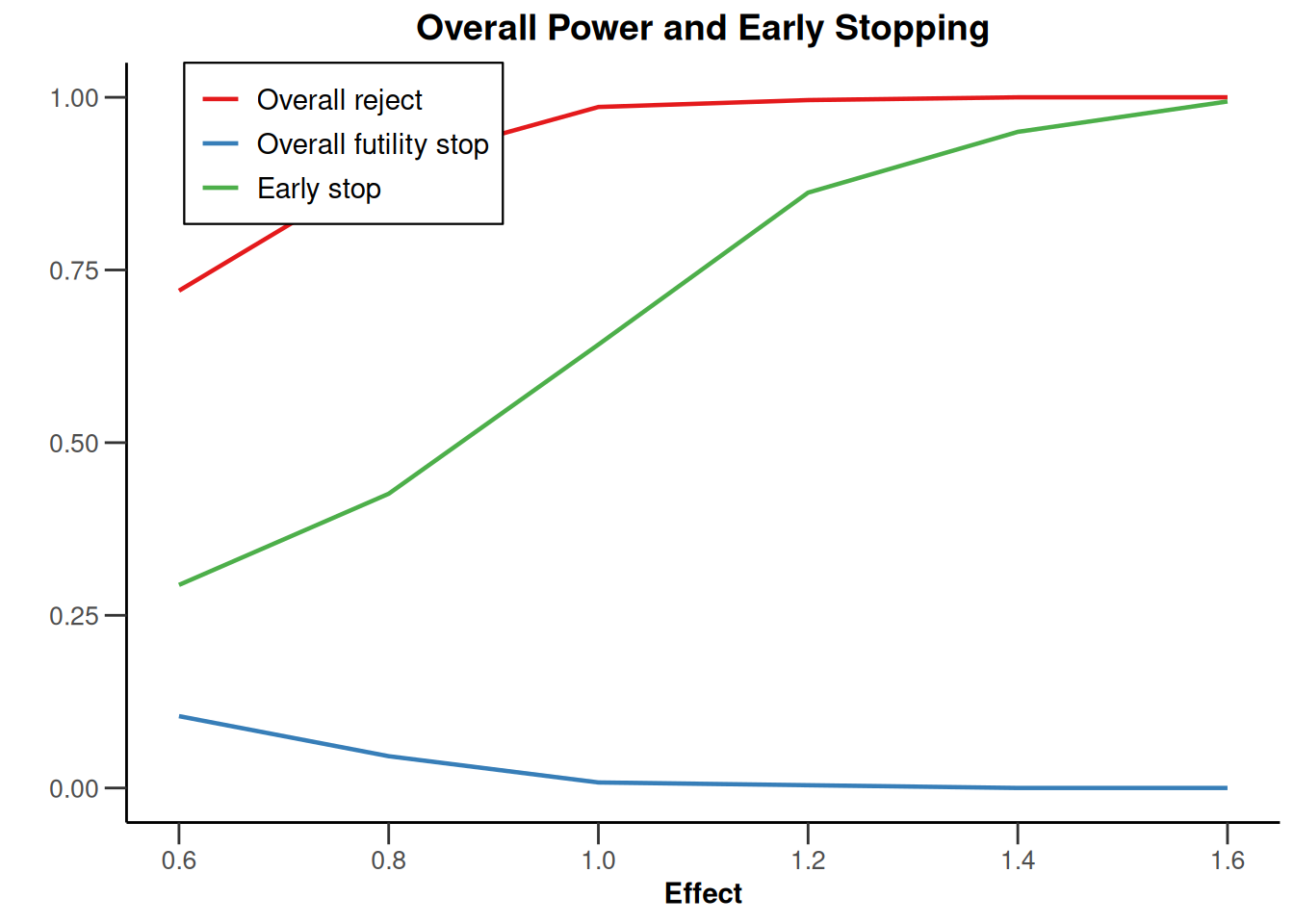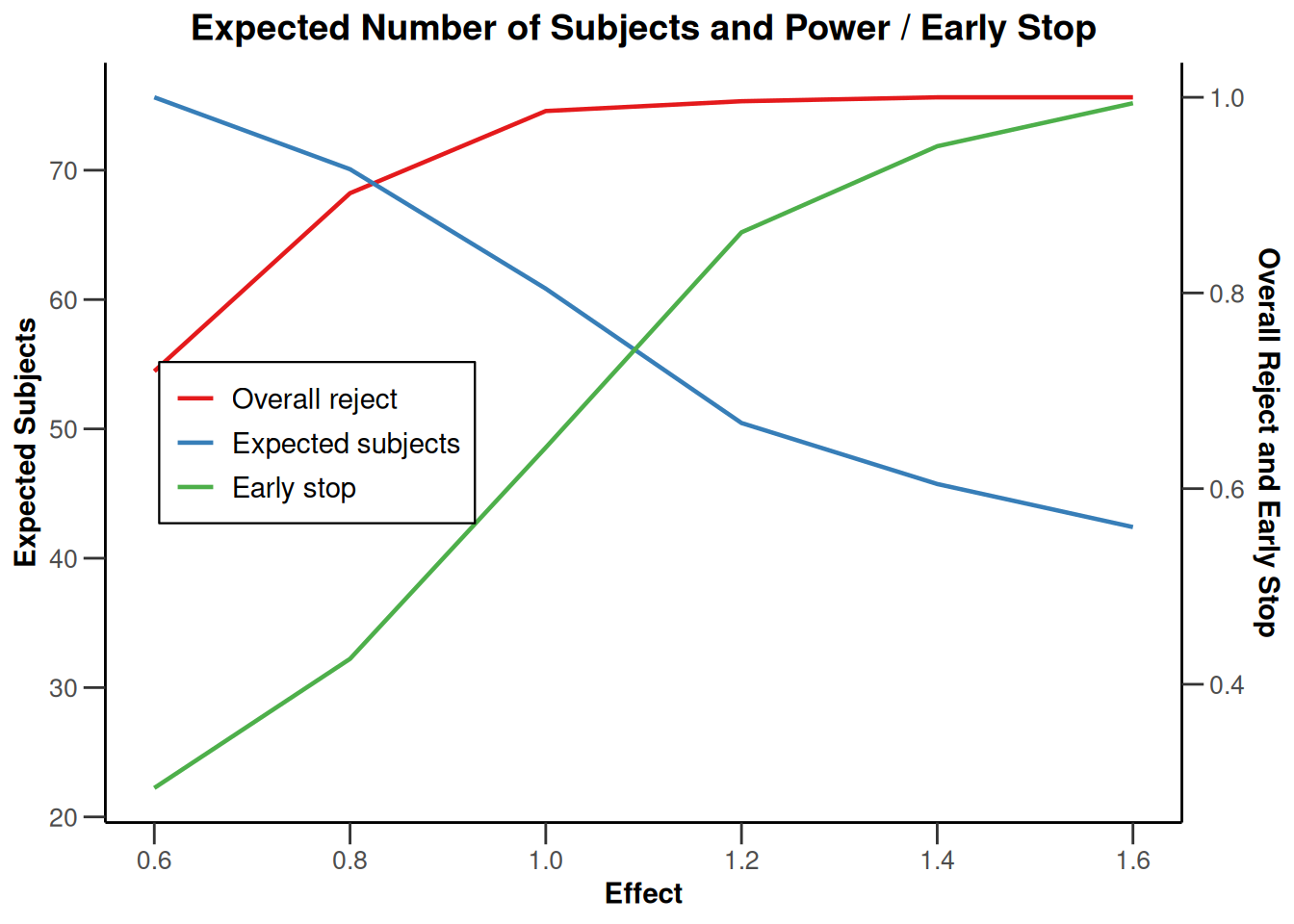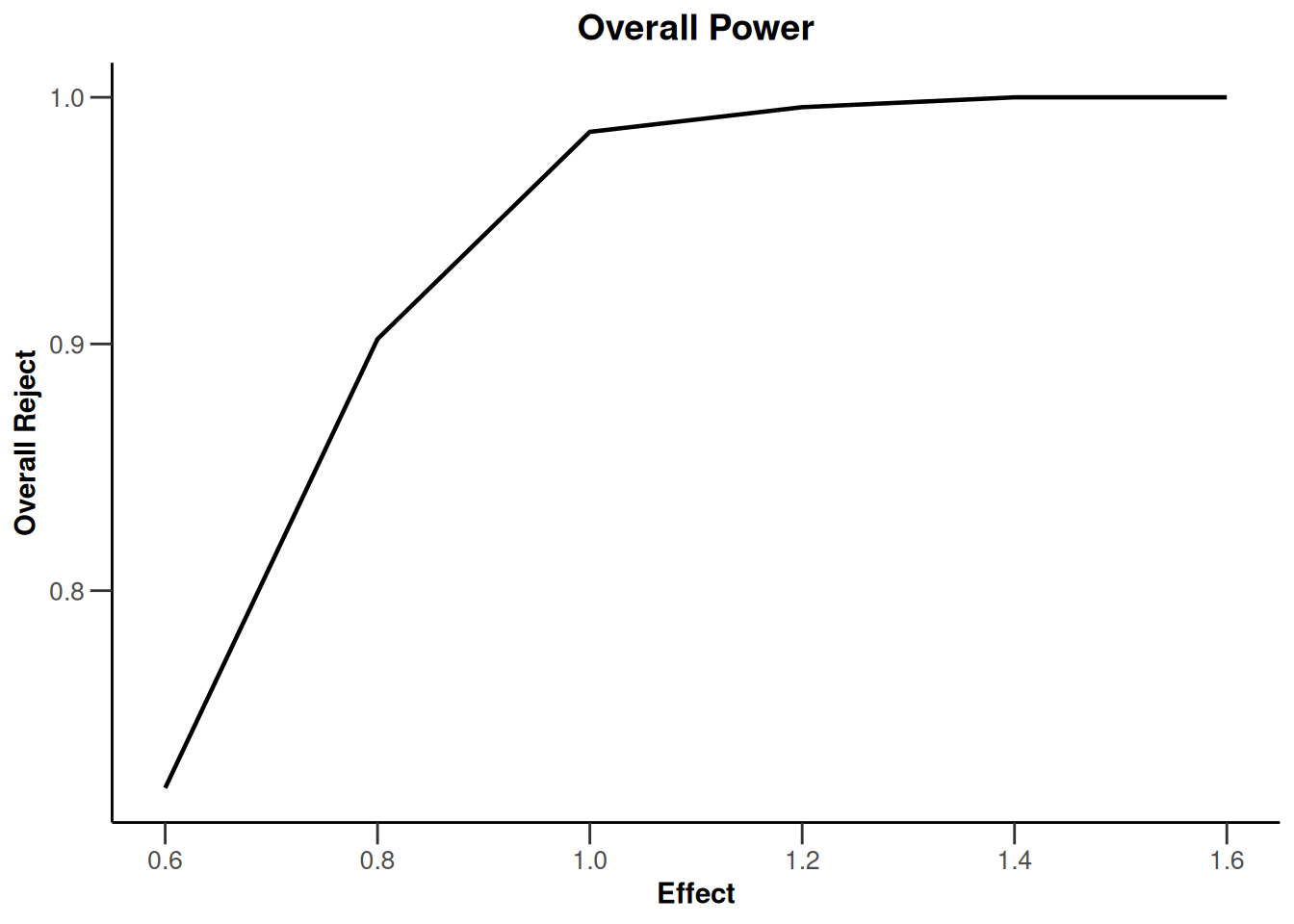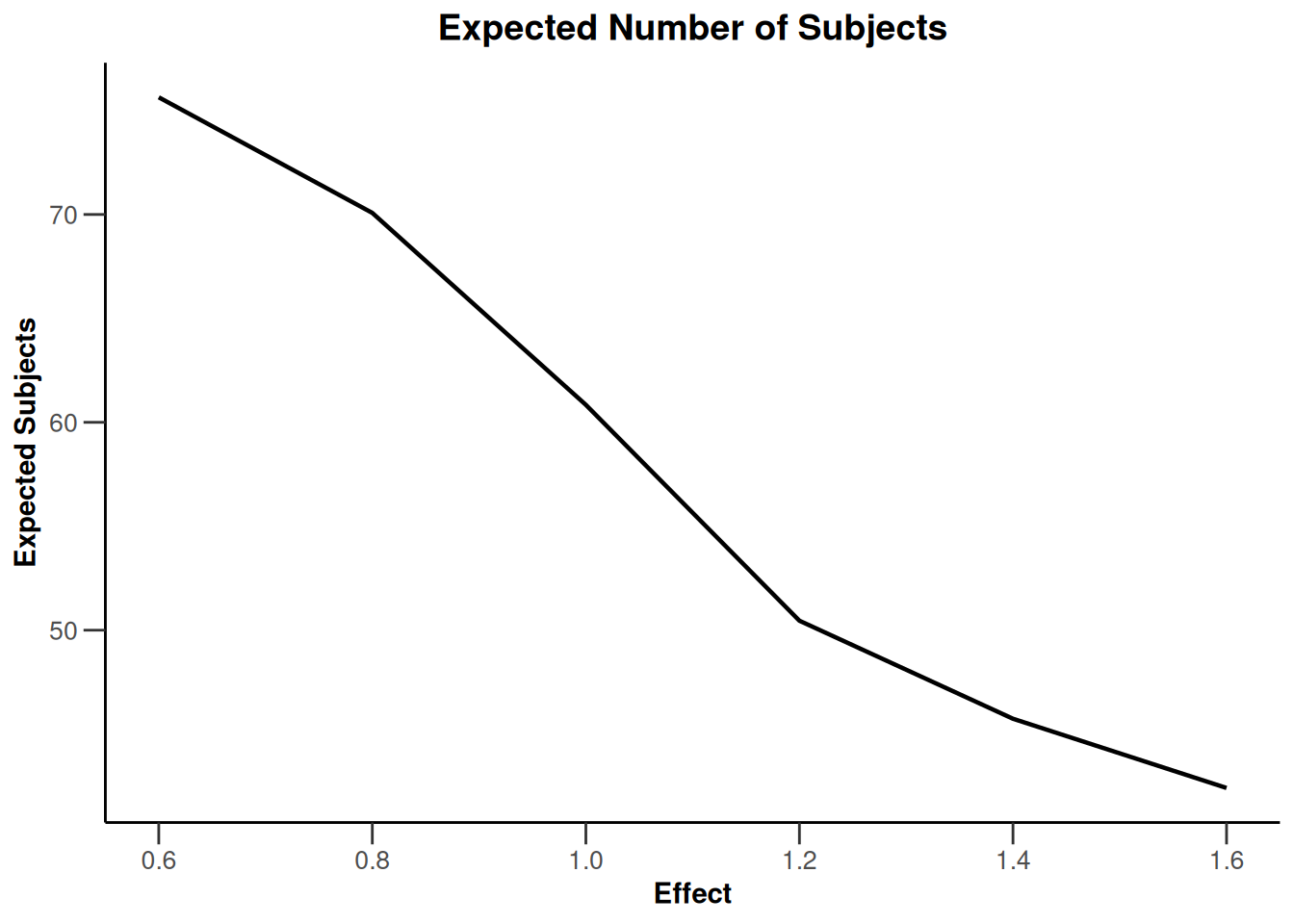## Simulation results base - rates

``````maxNumberOfSubjects <- 90
informationRates <- (1:3) / 3
plannedSubjects <- round(informationRates * maxNumberOfSubjects)
design <- getDesignInverseNormal(
futilityBounds = c(-0.5, 0.5),
informationRates = informationRates
)
x <- getSimulationRates(
design = getDesignFisher(),
groups = 2, riskRatio = TRUE,
thetaH0 = 0.8, plannedSubjects = plannedSubjects,
maxNumberOfIterations = 500, allocationRatioPlanned = 3,
seed = 1234567890
)
plot(x, type = "all", grid = 0)``````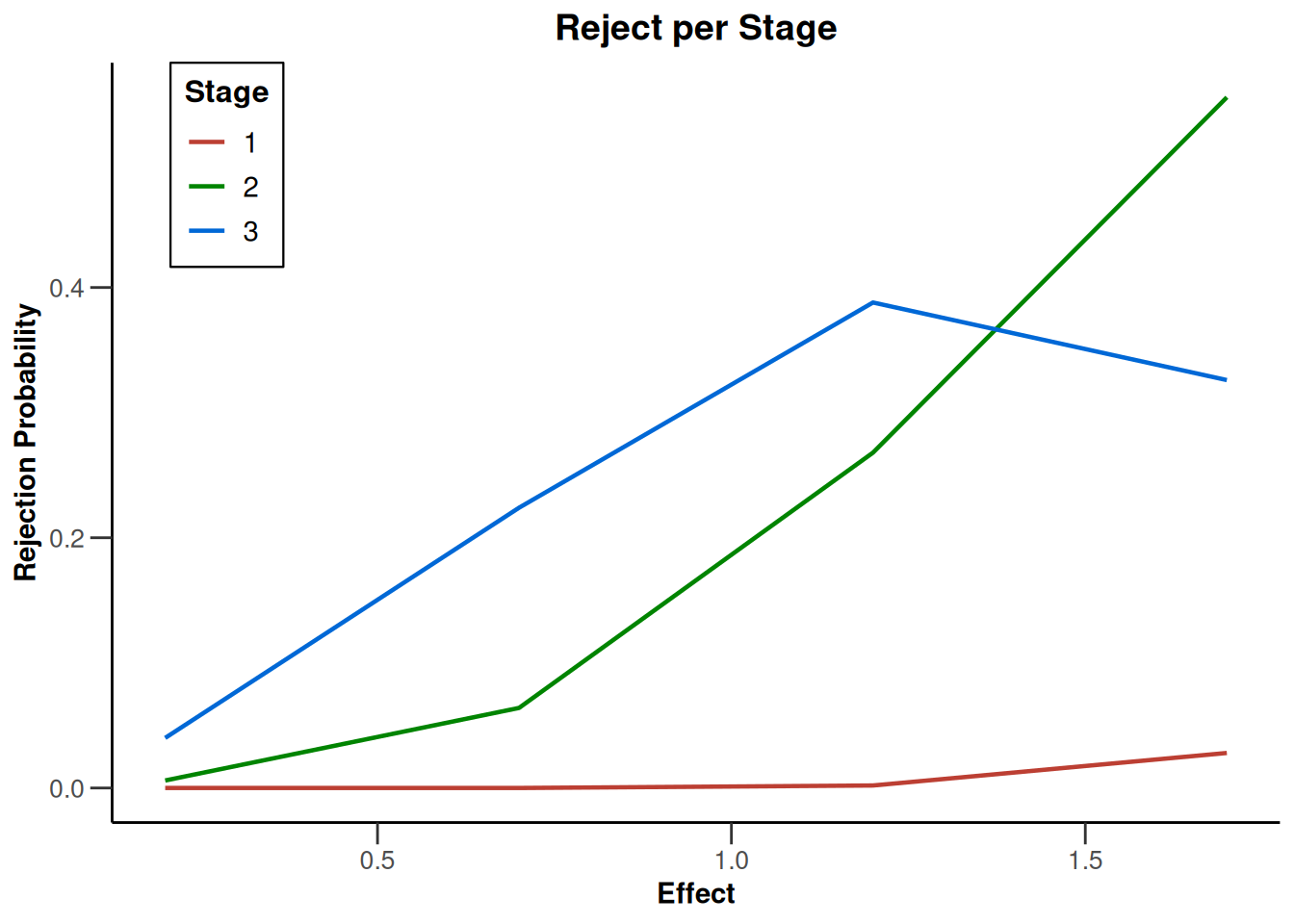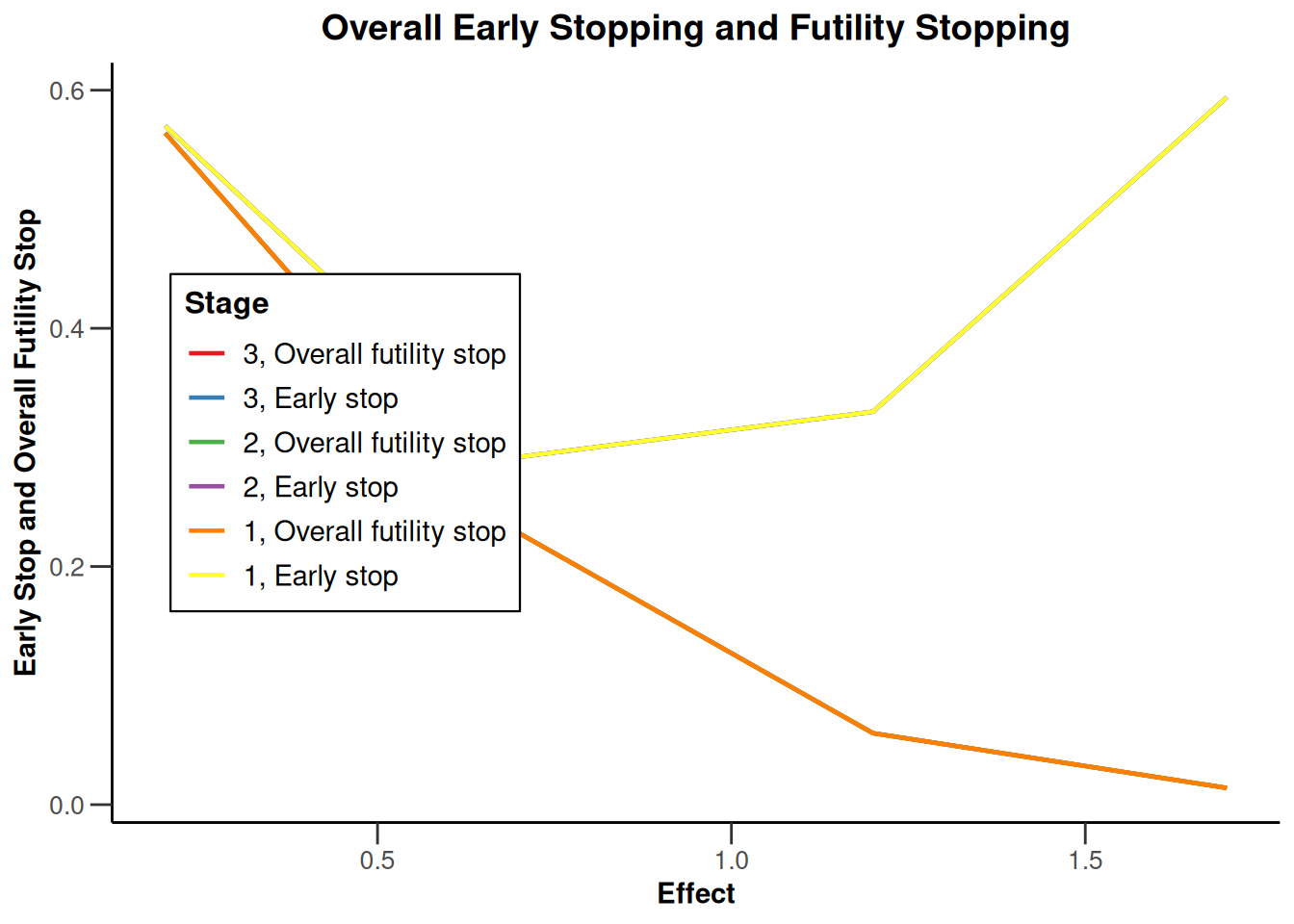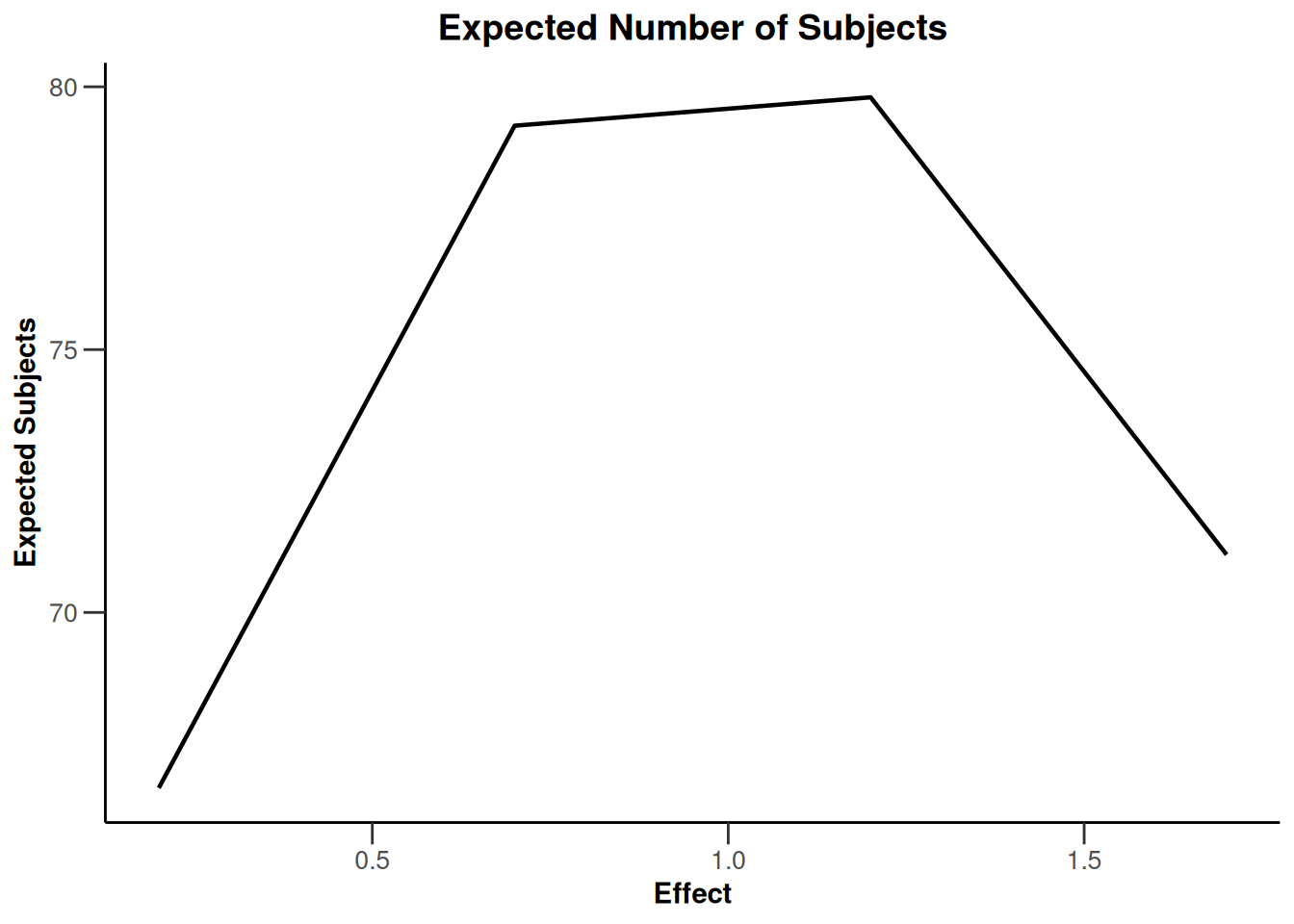## Simulation results base - survival

``````design <- getDesignFisher(kMax = 3, alpha0Vec = c(0.5, 0.5))
x <- getSimulationSurvival(
design = design, pi2 = 0.6,
pi1 = seq(0.3, 0.45, 0.05), directionUpper = FALSE,
maxNumberOfSubjects = 500, plannedEvents = (1:design\$kMax) * 20,
allocation1 = 1, allocation2 = 1, accrualTime = c(0, 3, 6, 12),
accrualIntensity = c(0.1, 0.2, 0.2), dropoutRate1 = 0,
dropoutRate2 = 0, dropoutTime = 12, conditionalPower = 0.8,
minNumberOfEventsPerStage = c(NA_real_, 10, 10),
maxNumberOfEventsPerStage = c(NA_real_, 100, 200),
maxNumberOfIterations = 500, seed = 1234567890
)
plot(x, type = "all", grid = 0)``````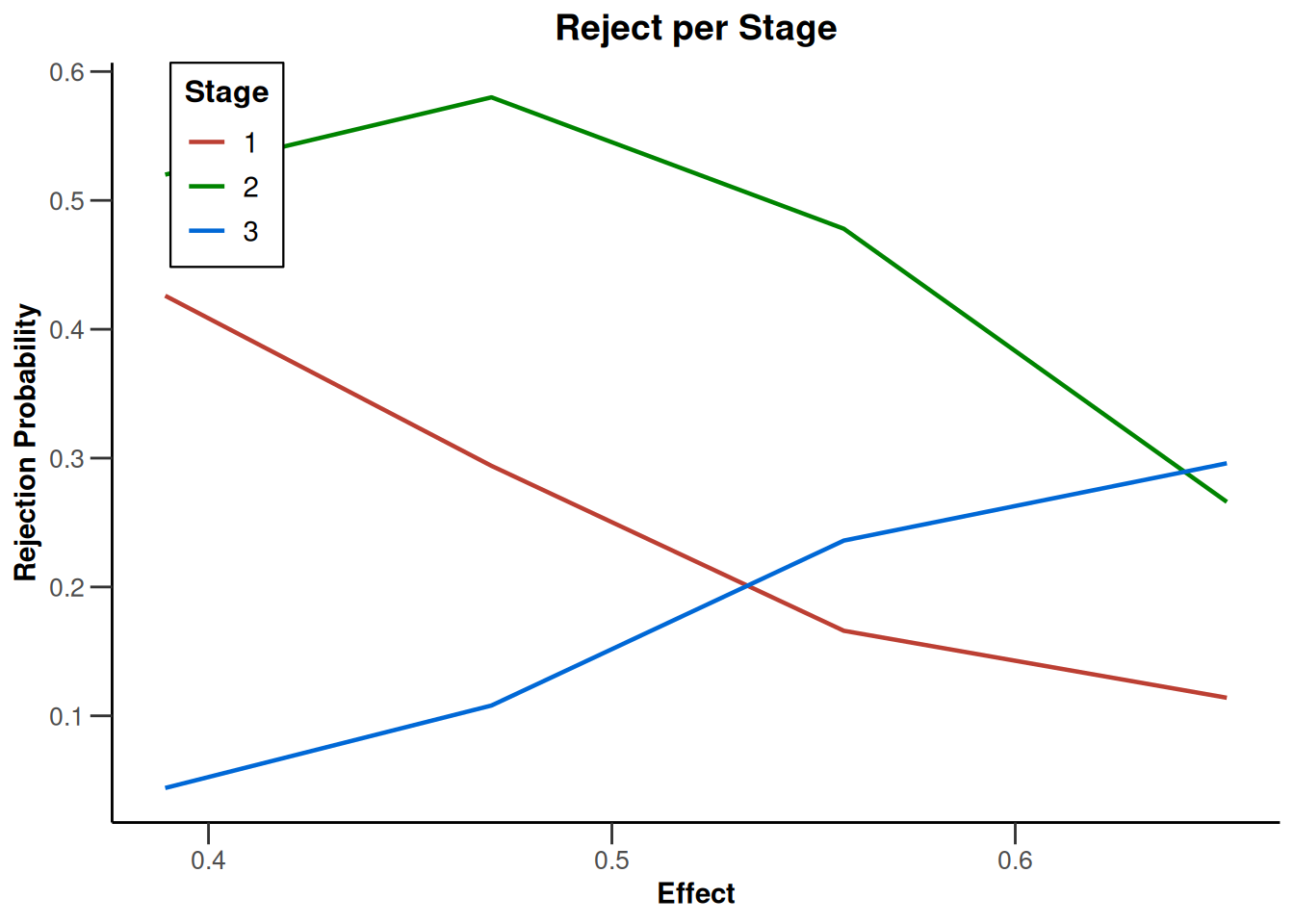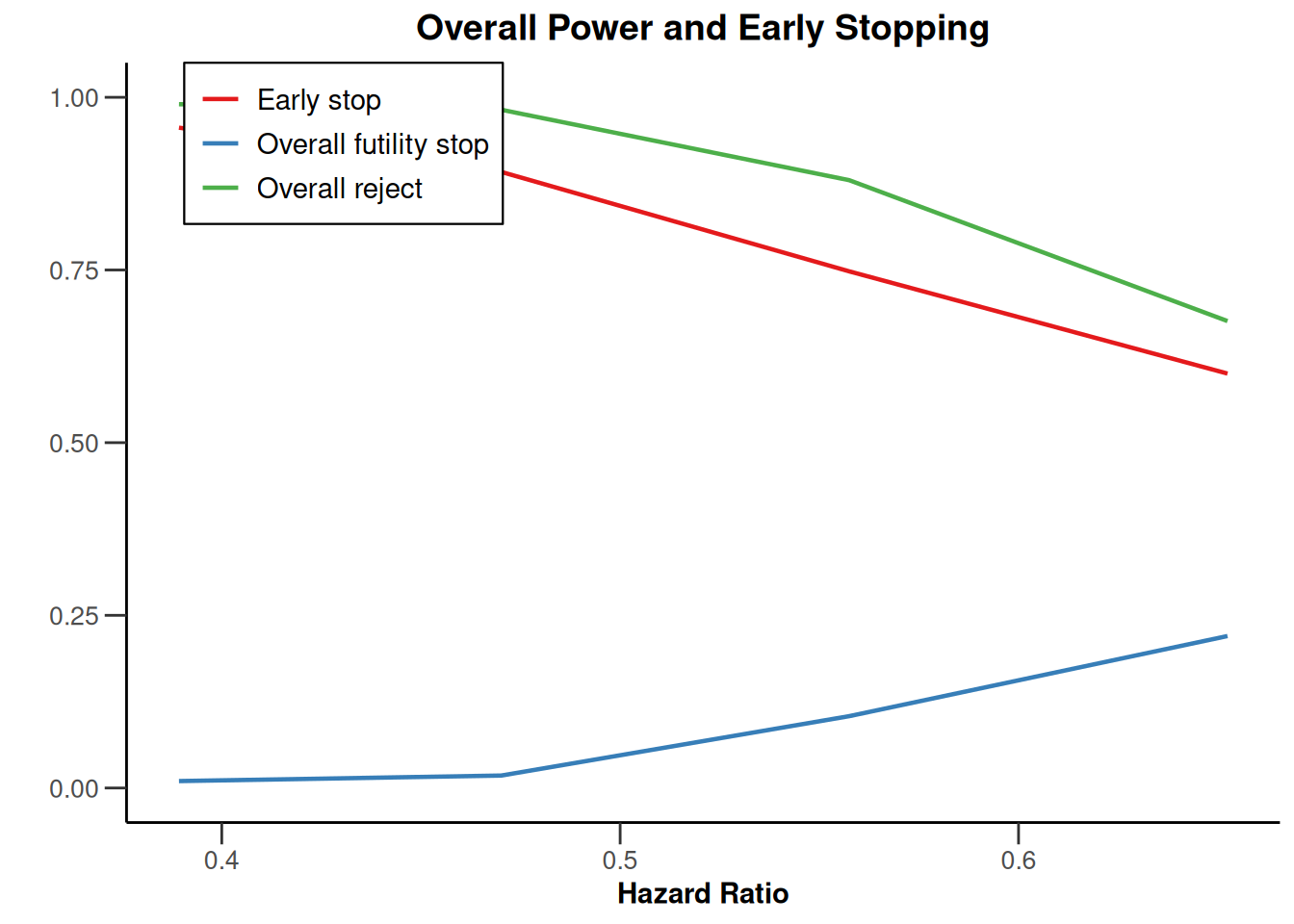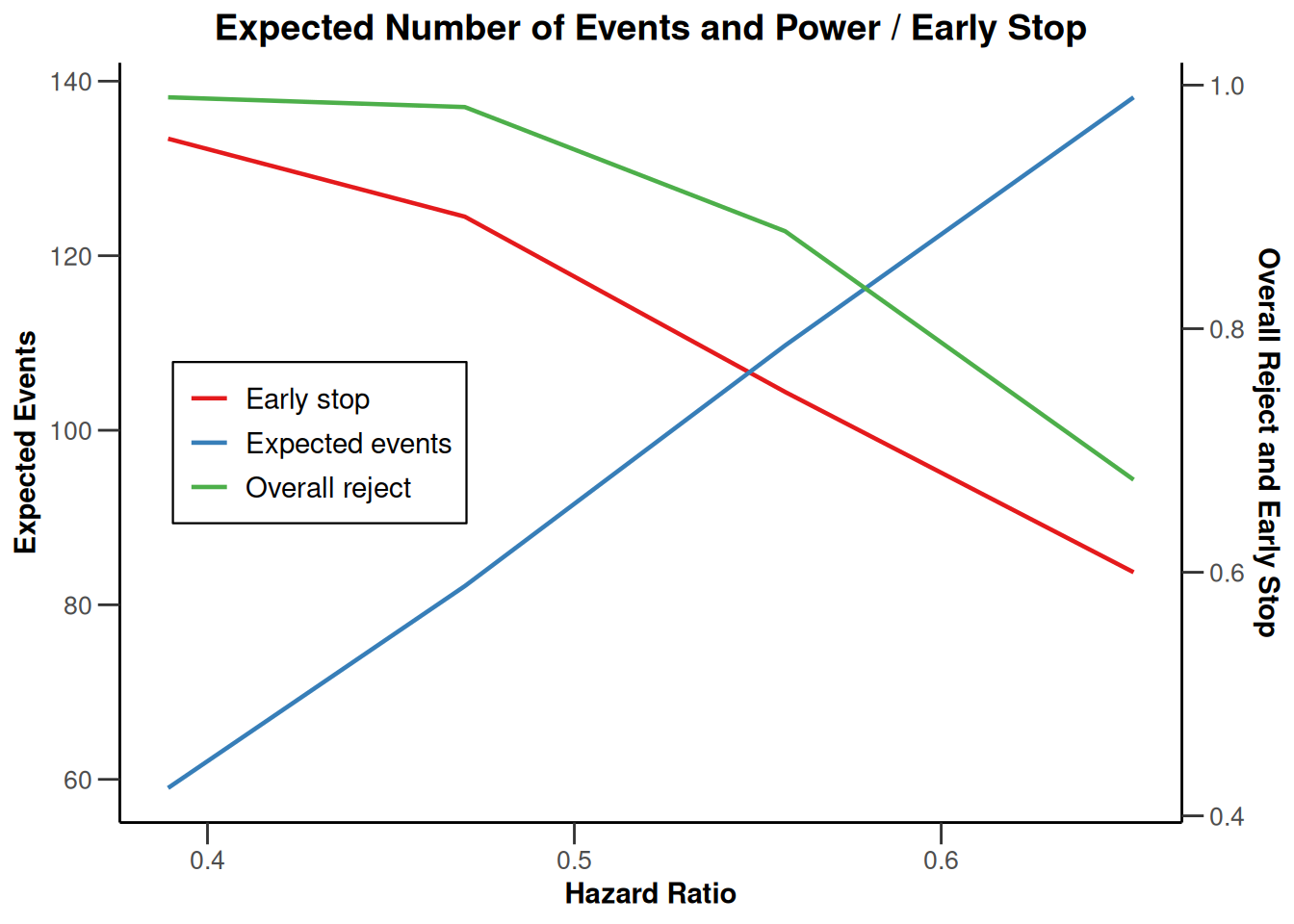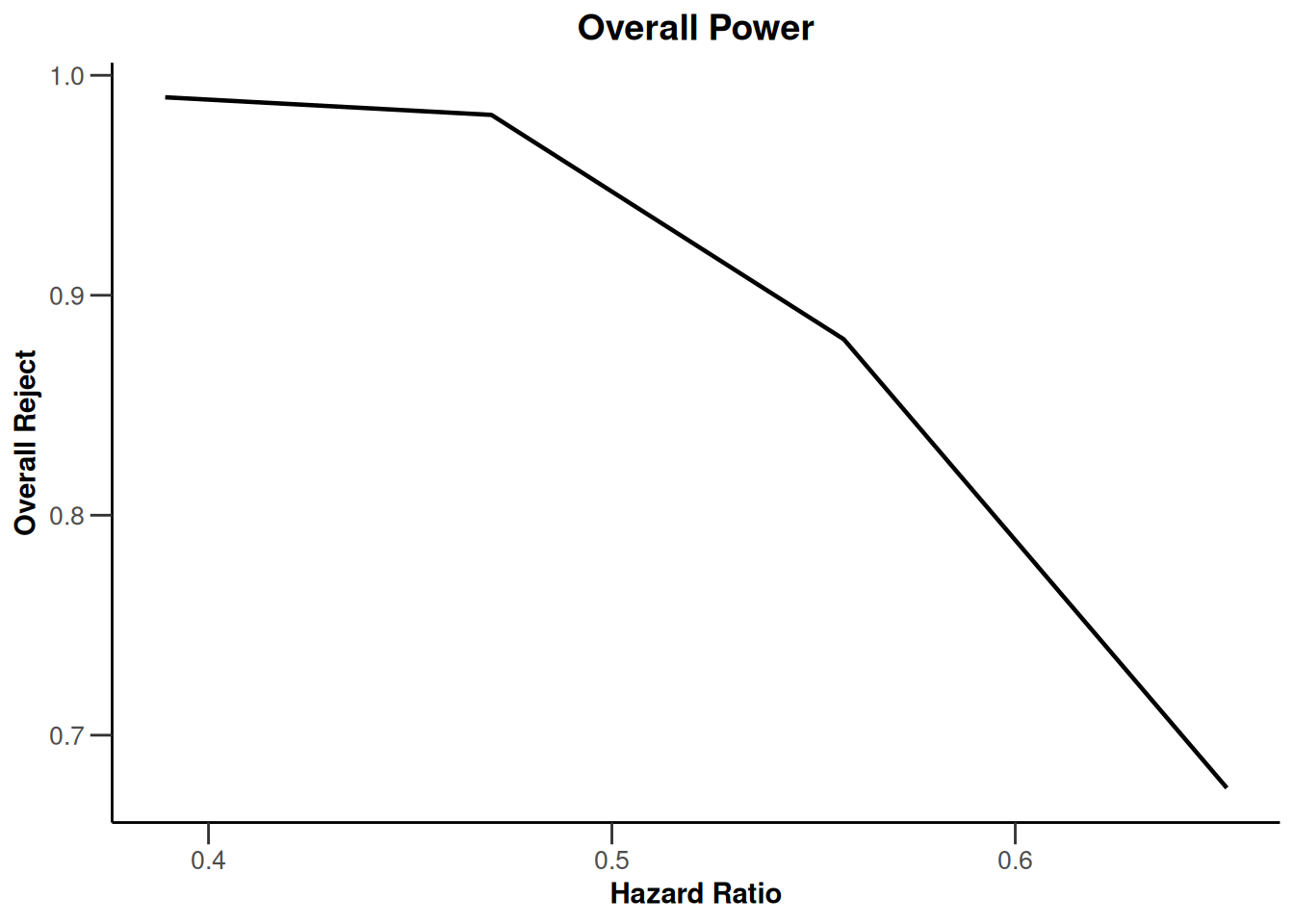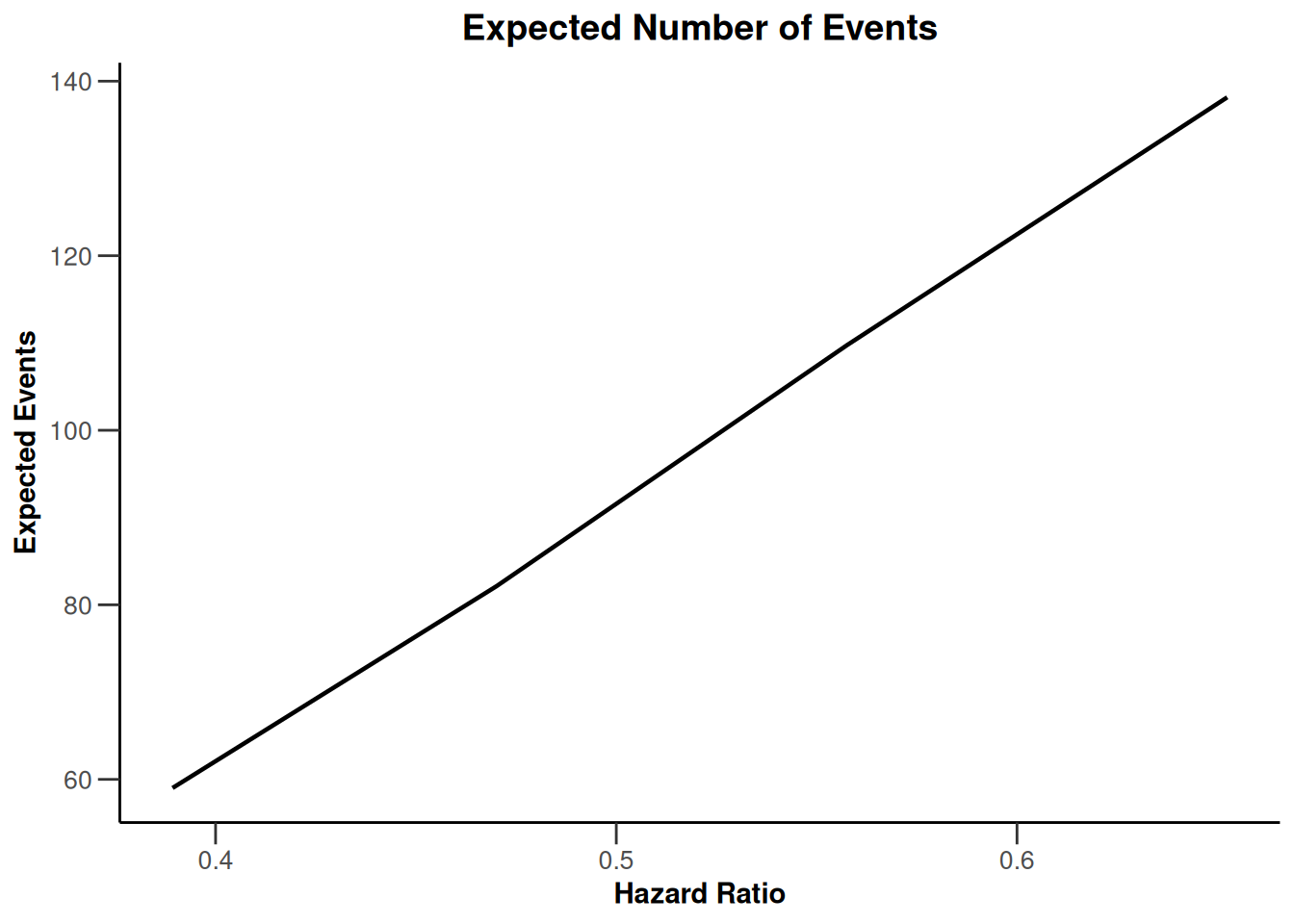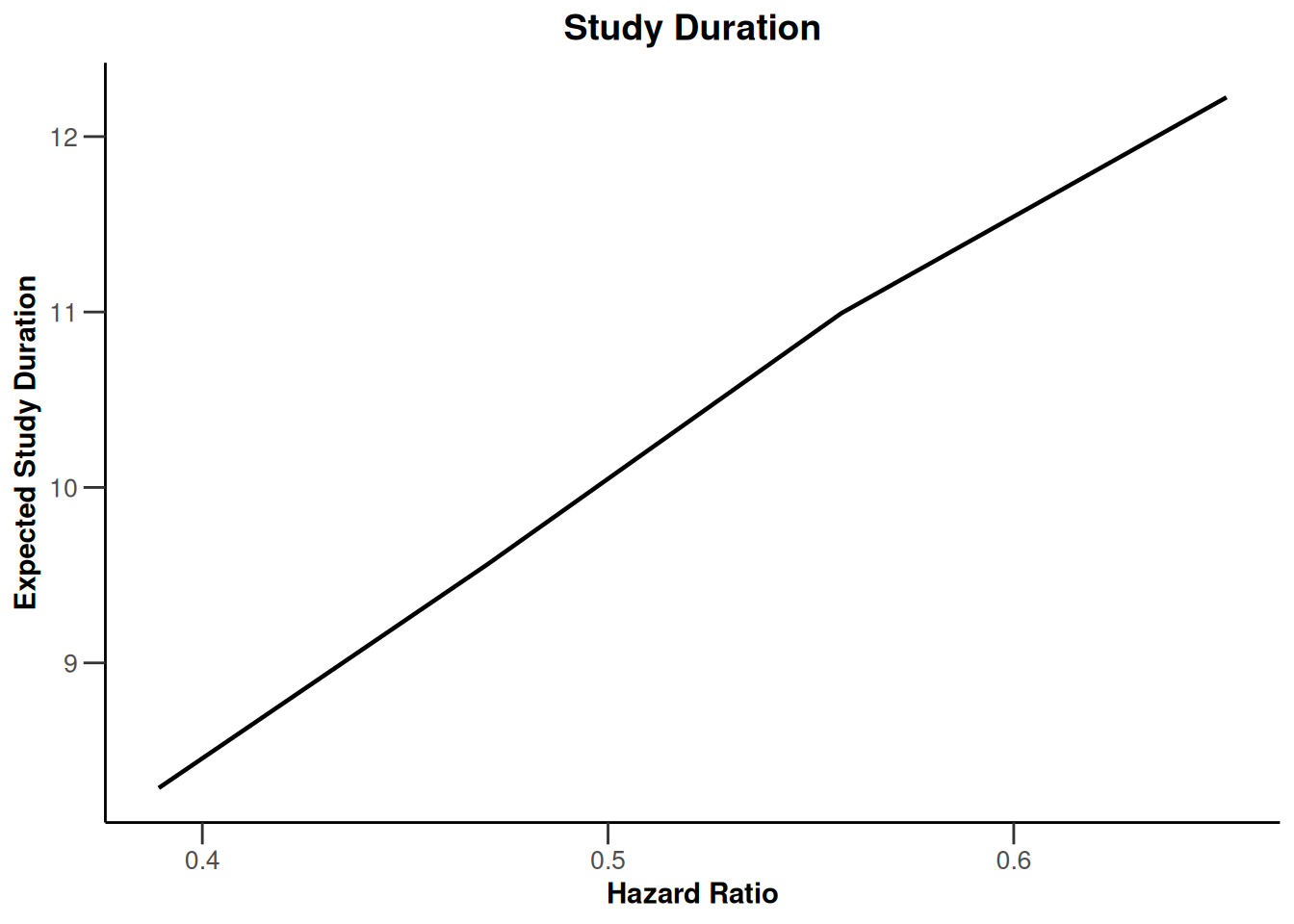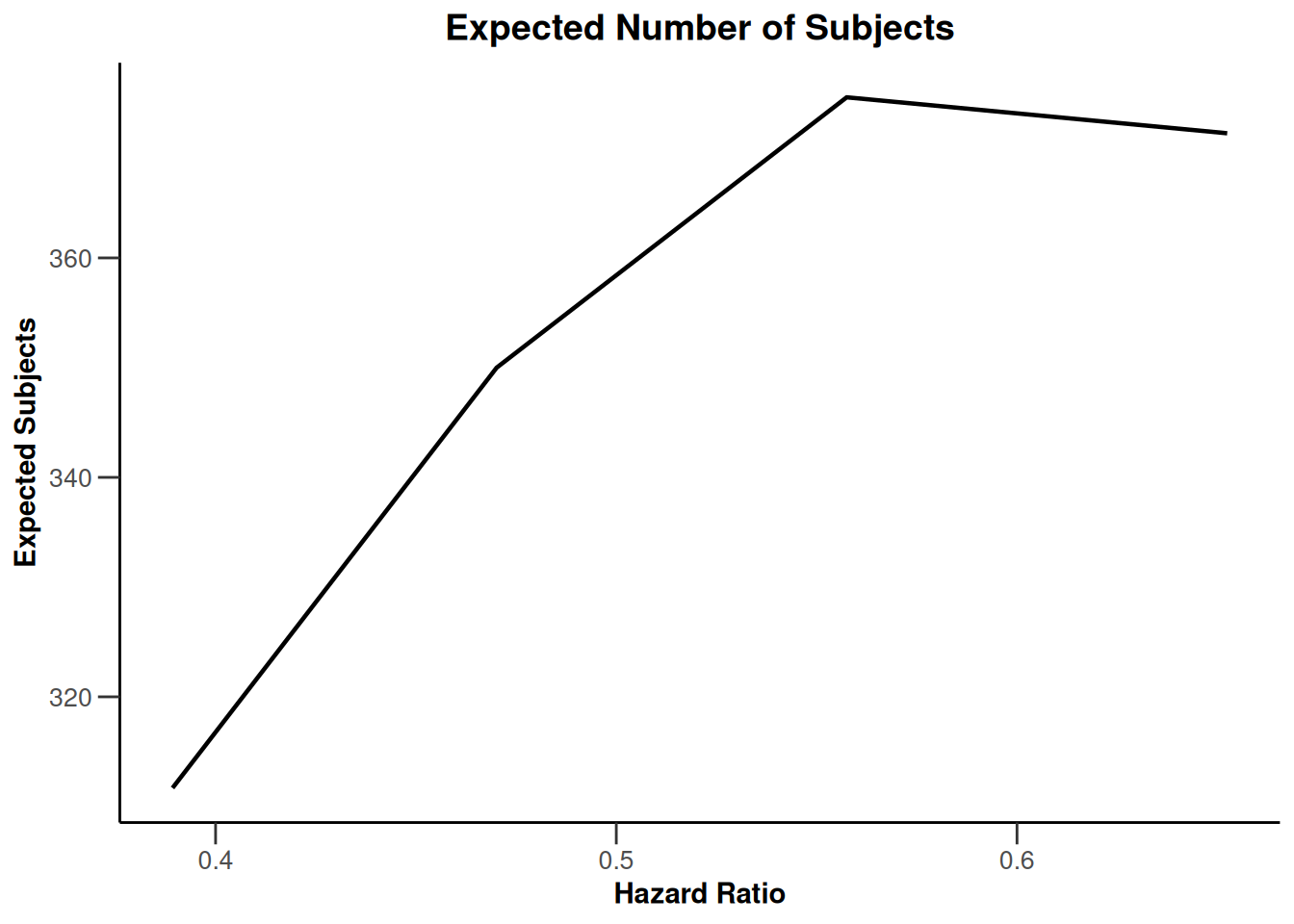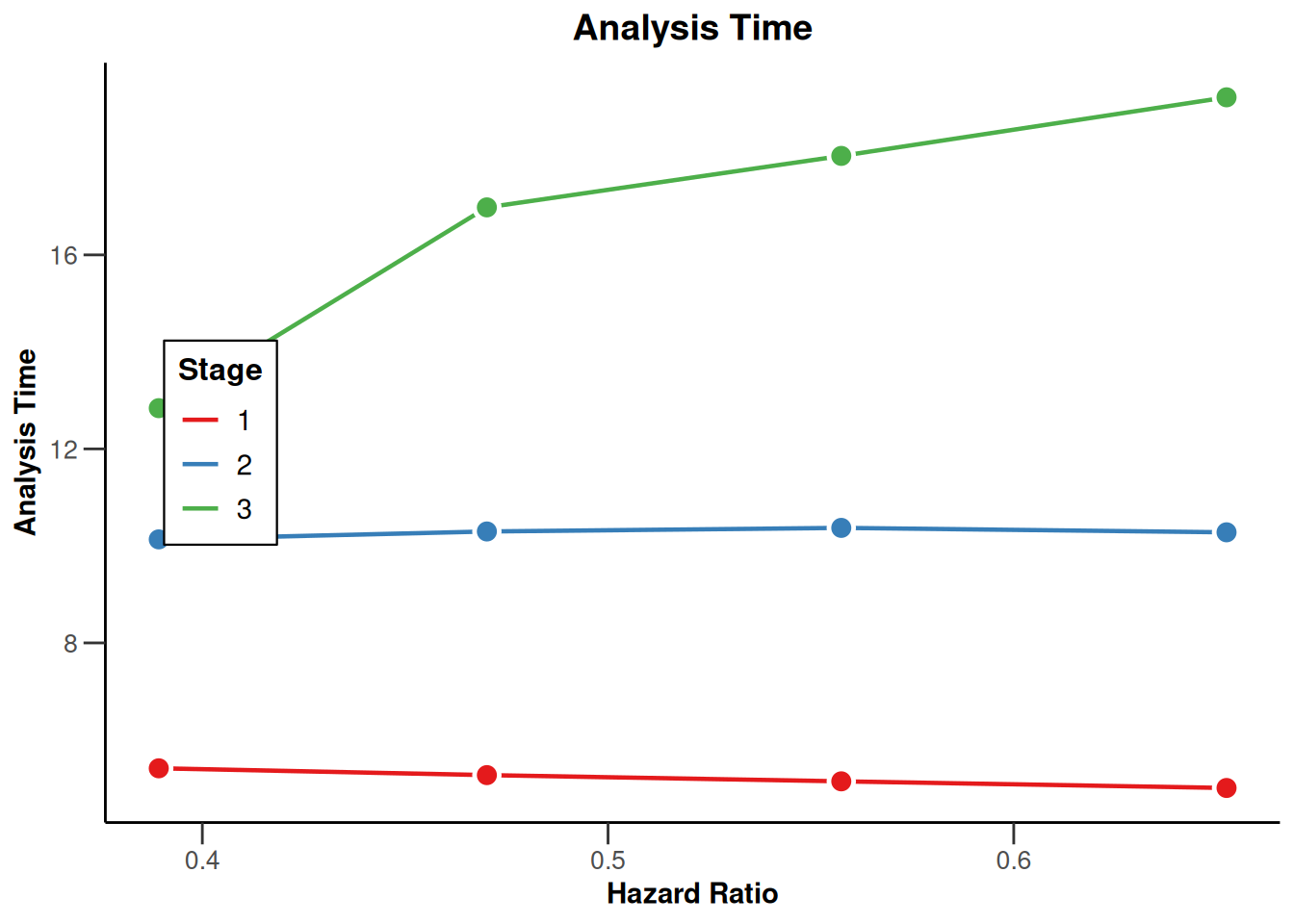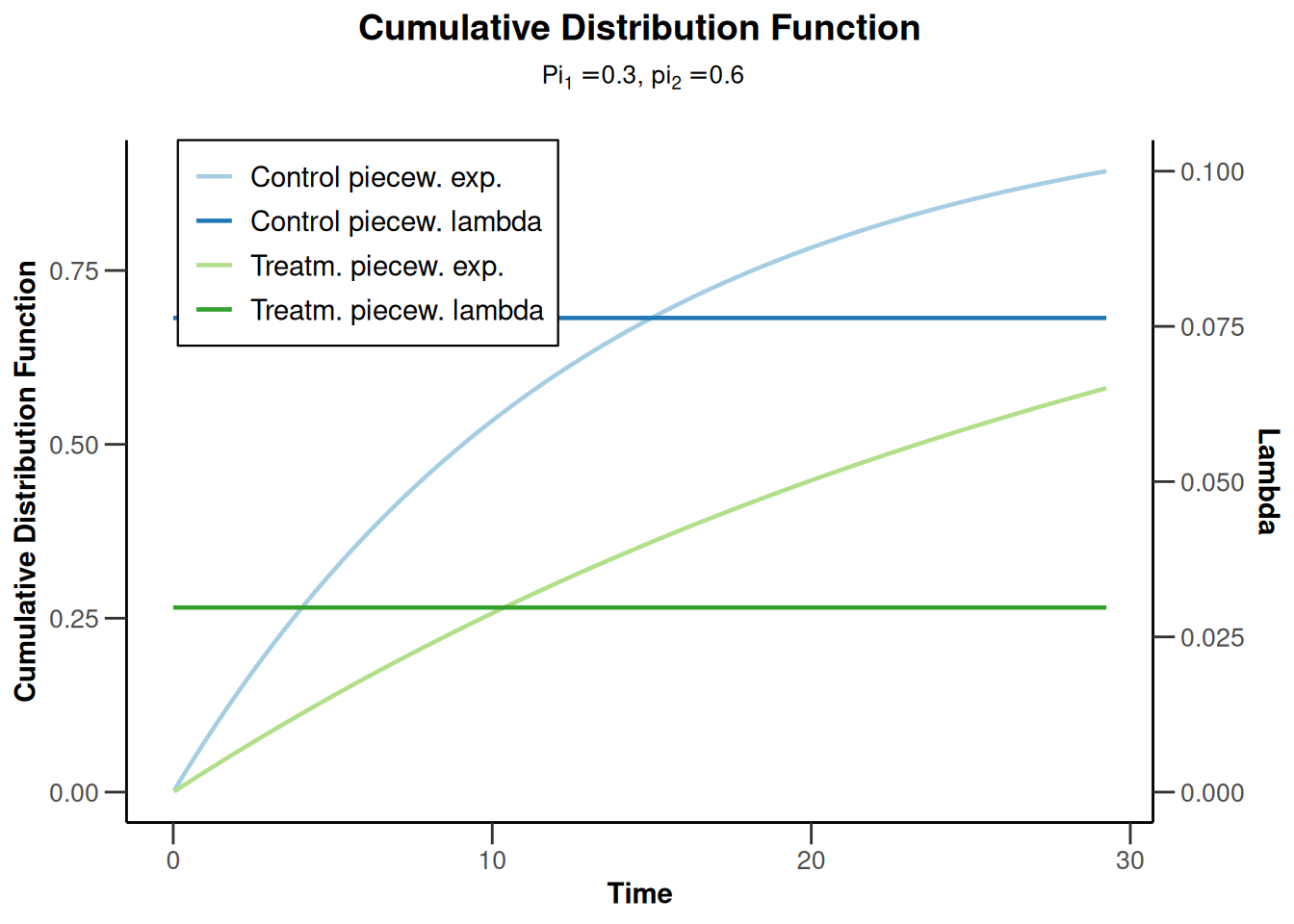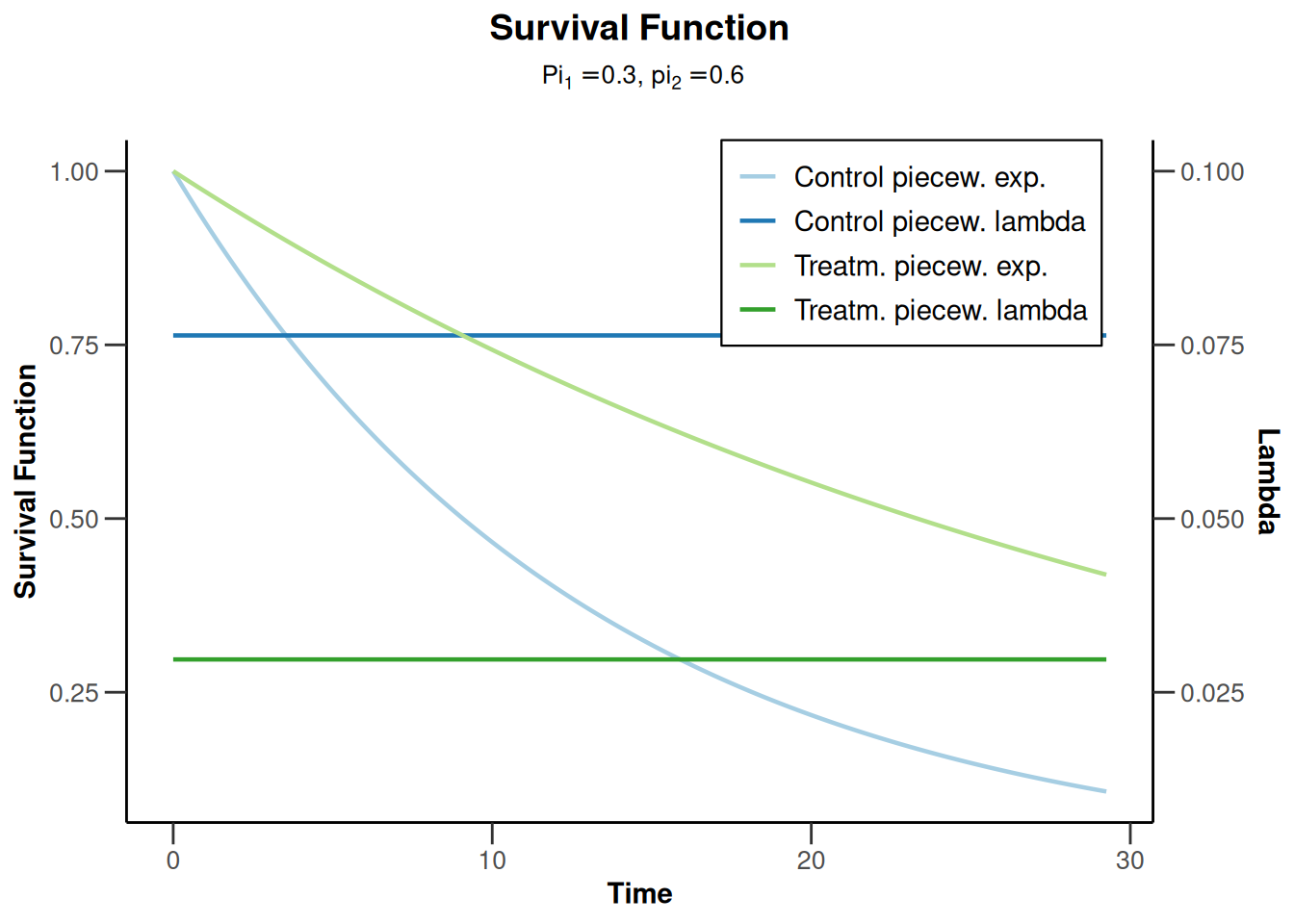``````design <- getDesignGroupSequential(kMax = 3, typeOfDesign = "WT", deltaWT = 0.25)
piecewiseSurvivalTime <- list(
"<6" = 0.025,
"6 - <9" = 0.04,
"9 - <15" = 0.015,
"15 - <21" = 0.01,
">=21" = 0.007
)
x <- getSimulationSurvival(
design = design,
directionUpper = TRUE, maxNumberOfSubjects = 500,
plannedEvents = (1:design\$kMax) * 20, allocation1 = 1,
allocation2 = 1, accrualTime = c(0, 3, 6, 12),
piecewiseSurvivalTime = piecewiseSurvivalTime, hazardRatio = 1.7,
accrualIntensity = c(0.1, 0.2, 0.2), dropoutRate1 = 0,
dropoutRate2 = 0, dropoutTime = 12, conditionalPower = 0.8,
minNumberOfEventsPerStage = c(NA_real_, 10, 10),
maxNumberOfEventsPerStage = c(NA_real_, 100, 200),
maxNumberOfIterations = 500, seed = 1234567890
)
plot(x, type = "all", grid = 0)``````
``````Warning in !is.null(lambda1) && !is.na(lambda1): 'length(x) = 5 > 1' in
coercion to 'logical(1)'

Warning in !is.null(lambda1) && !is.na(lambda1): 'length(x) = 5 > 1' in
coercion to 'logical(1)'

Warning in !is.null(lambda1) && !is.na(lambda1): 'length(x) = 5 > 1' in
coercion to 'logical(1)'

Warning in !is.null(lambda1) && !is.na(lambda1): 'length(x) = 5 > 1' in
coercion to 'logical(1)'``````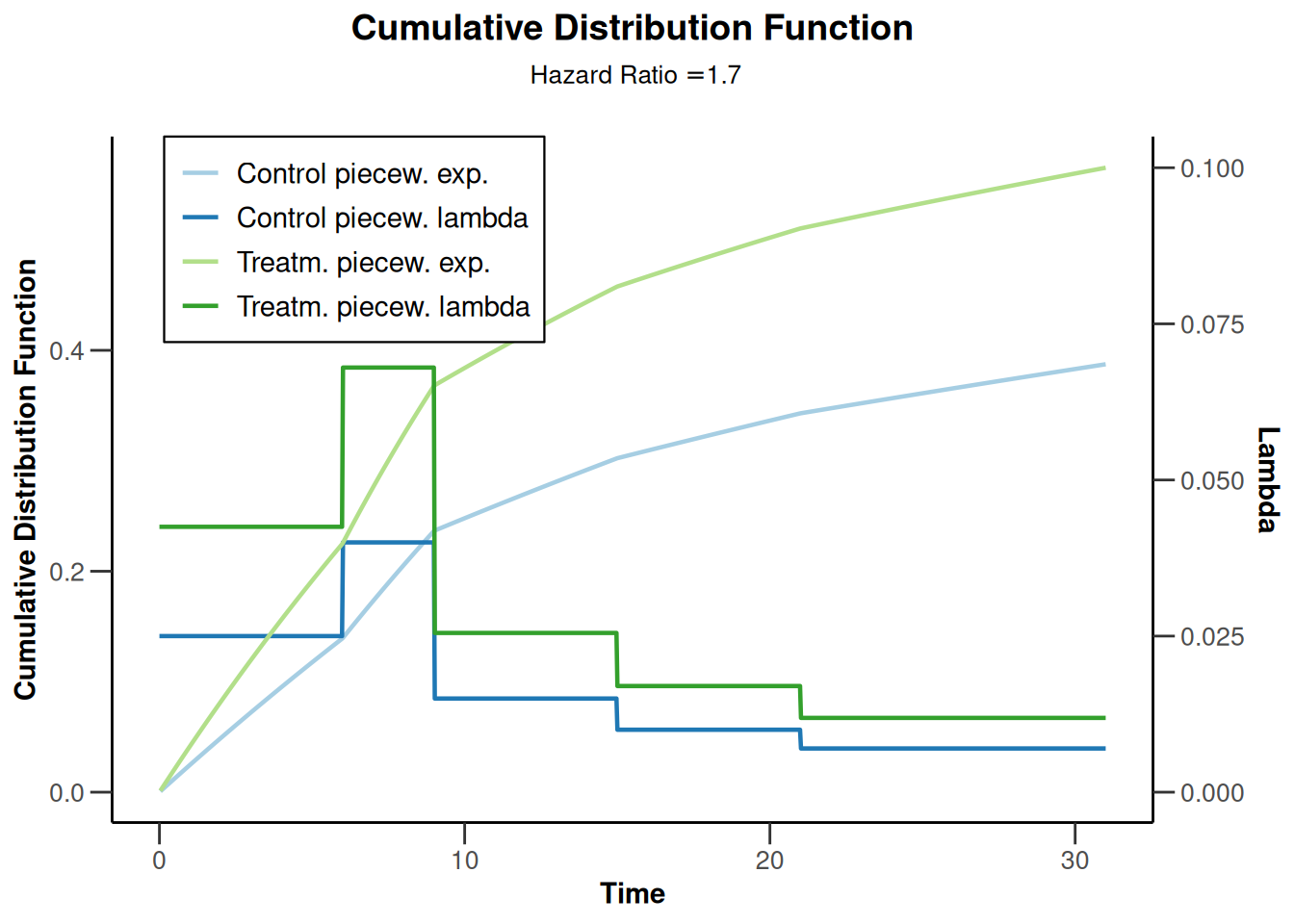# Simulation results multi-arm

## Simulation results multi-arm - means

``````design <- getDesignInverseNormal(
informationRates = c(0.2, 0.6, 1),
futilityBounds = c(-0.5, 0.5)
)
x <- getSimulationMultiArmMeans(
design = design, typeOfShape = "linear",
activeArms = 4, plannedSubjects = c(10, 30, 50), stDev = 1.2,
muMaxVector = seq(0.3, 0.6, 0.1), adaptations = rep(TRUE, 2),
conditionalPower = 0.8, minNumberOfSubjectsPerStage = c(10, 4, 4),
maxNumberOfSubjectsPerStage = c(10, 100, 100),
maxNumberOfIterations = 500, seed = 1234567890
)
plot(x, type = "all", grid = 0)``````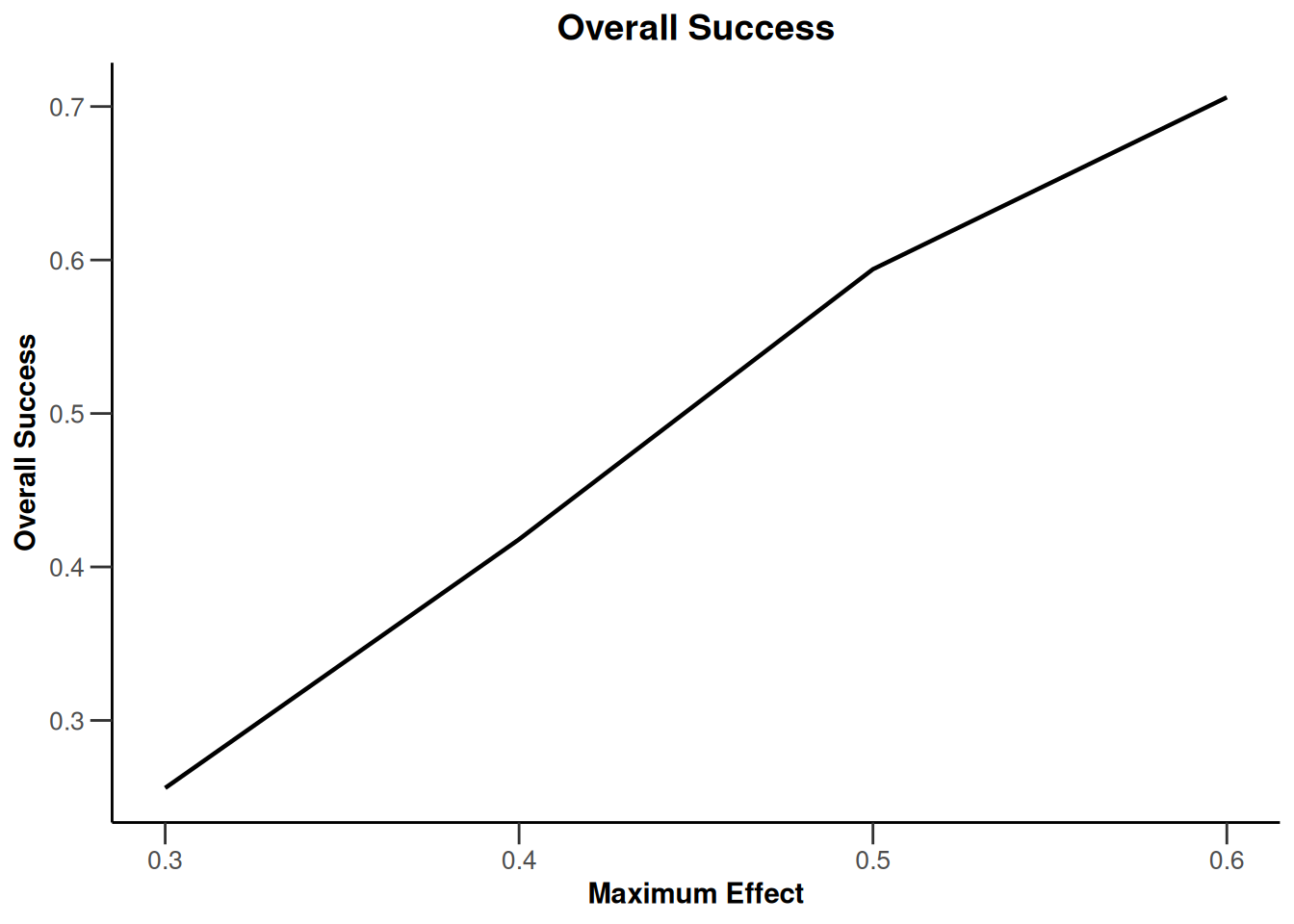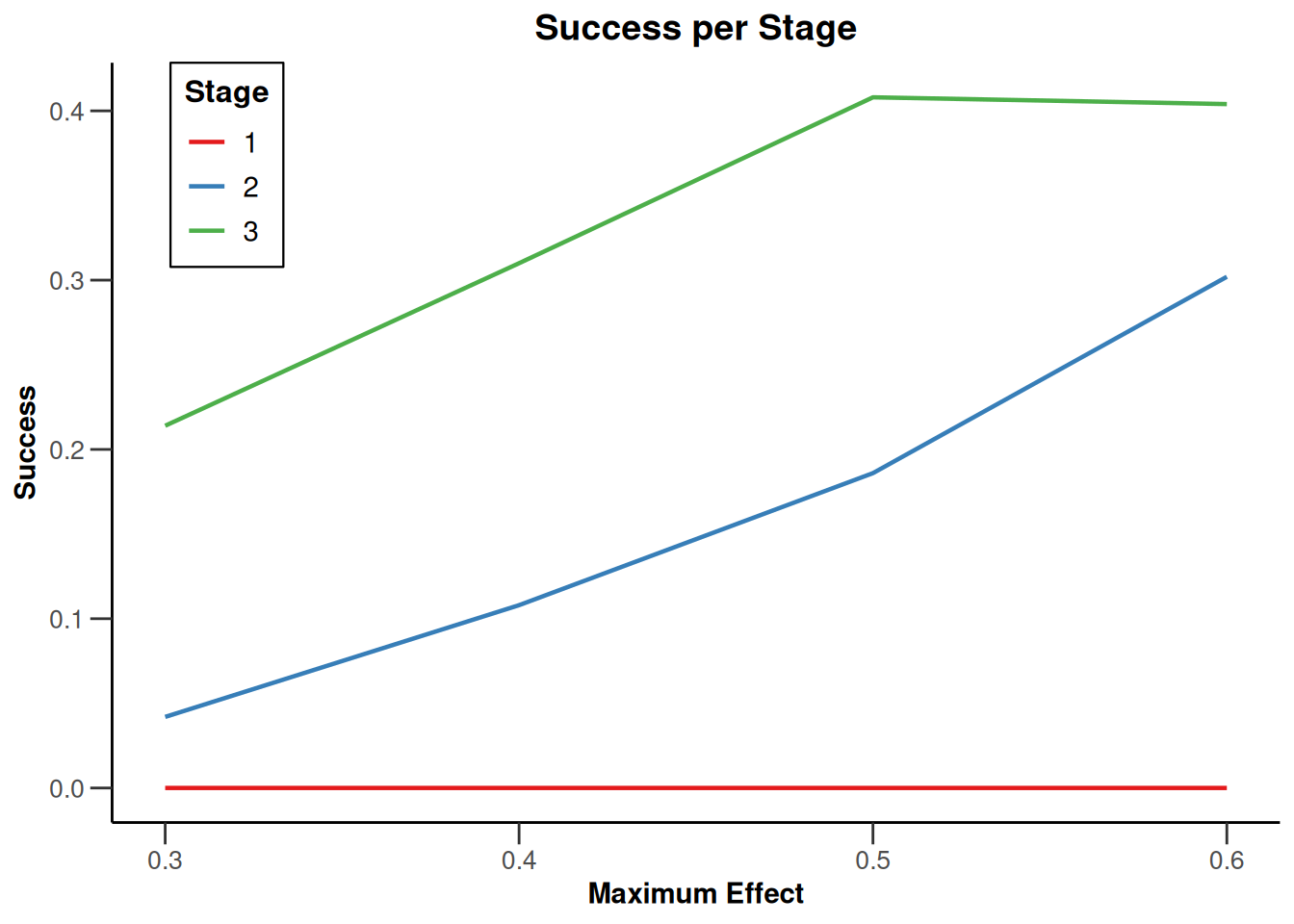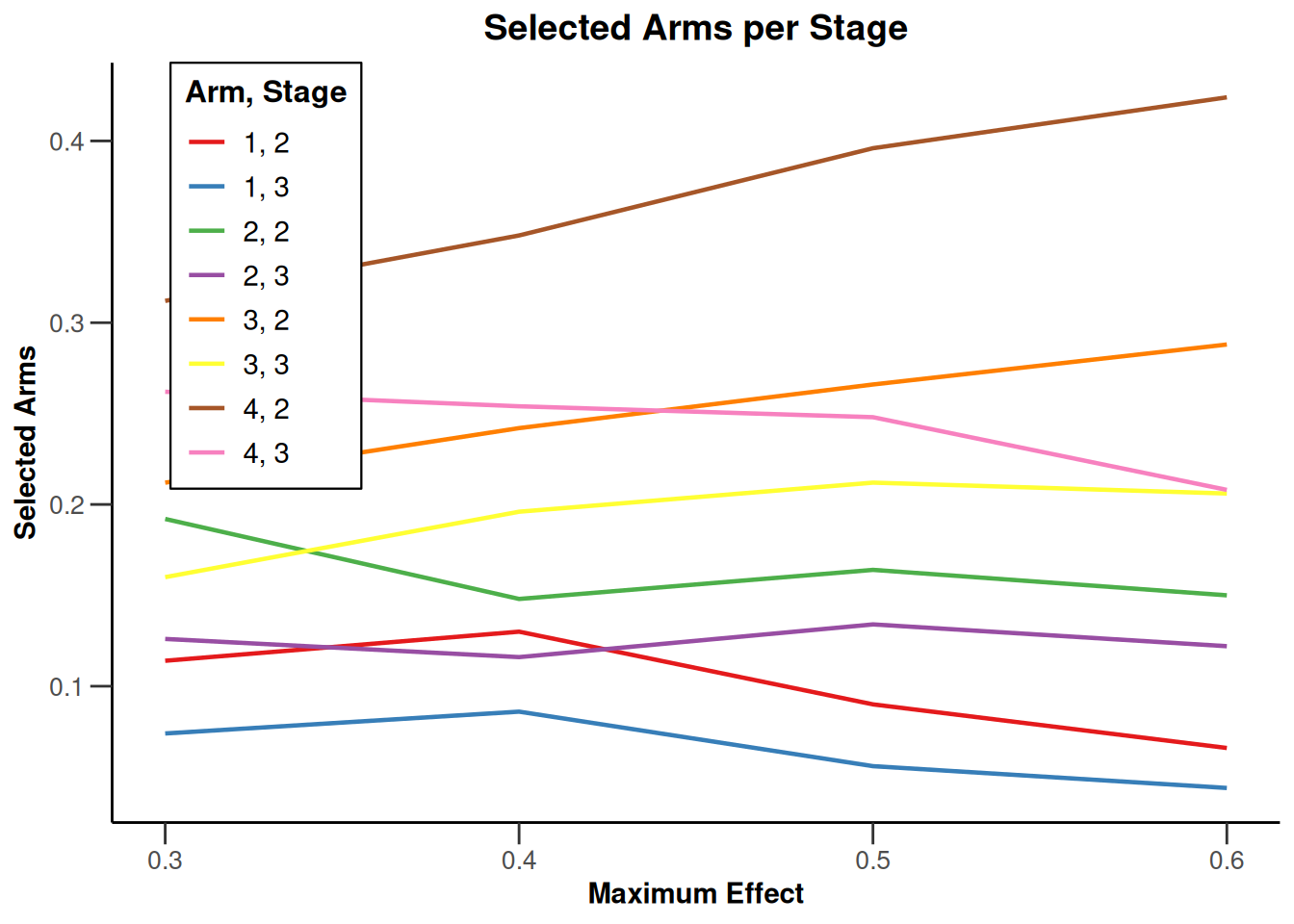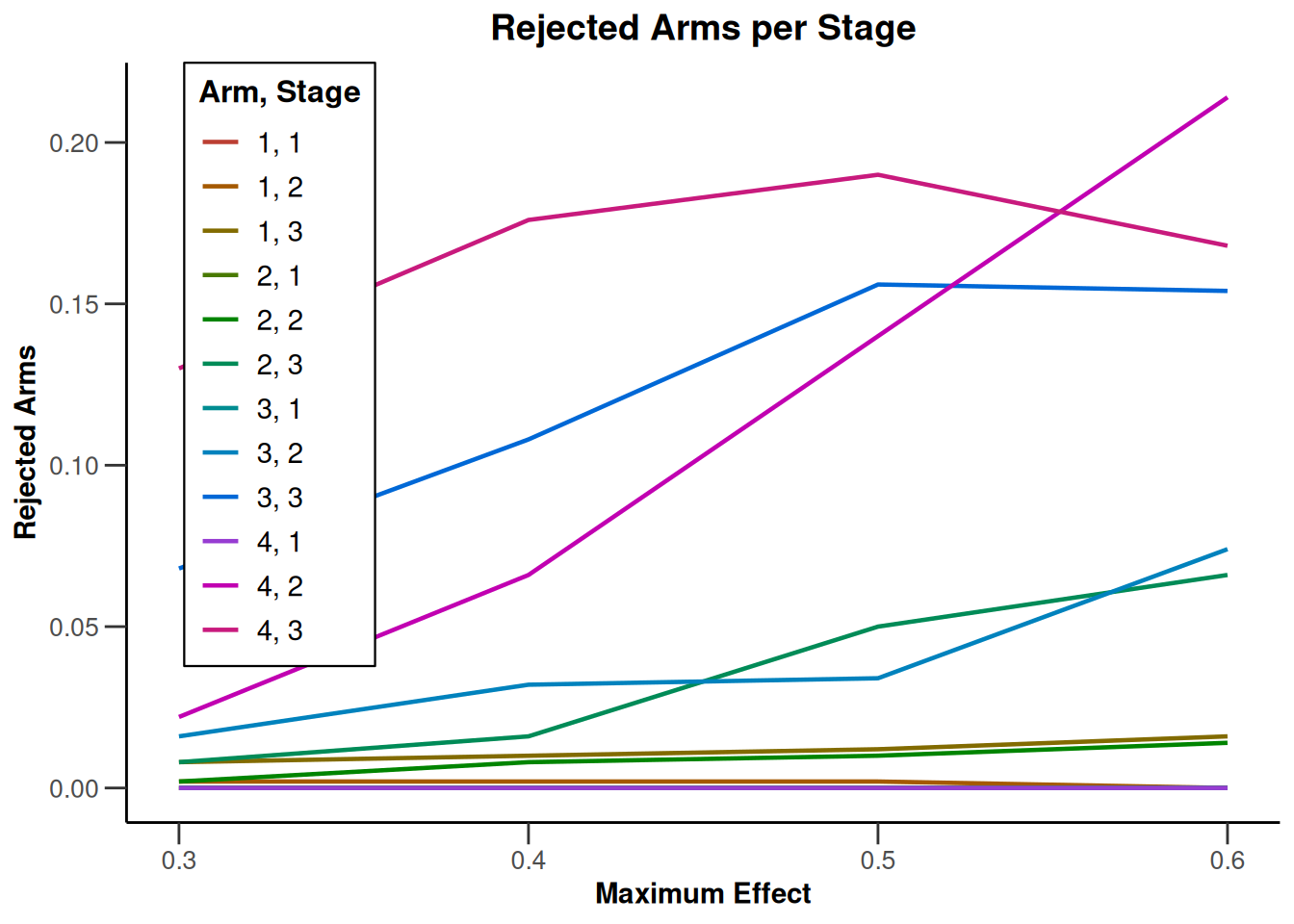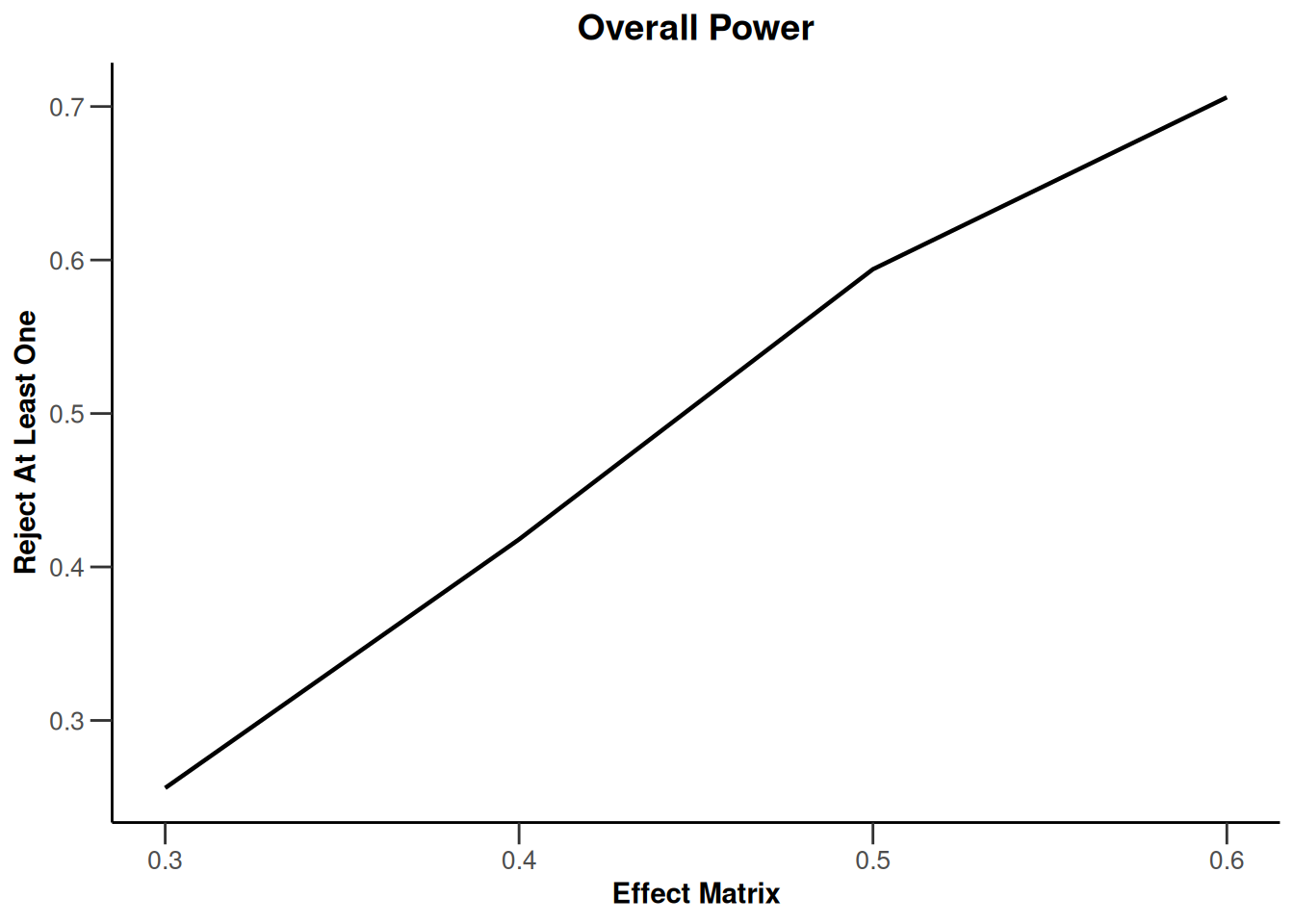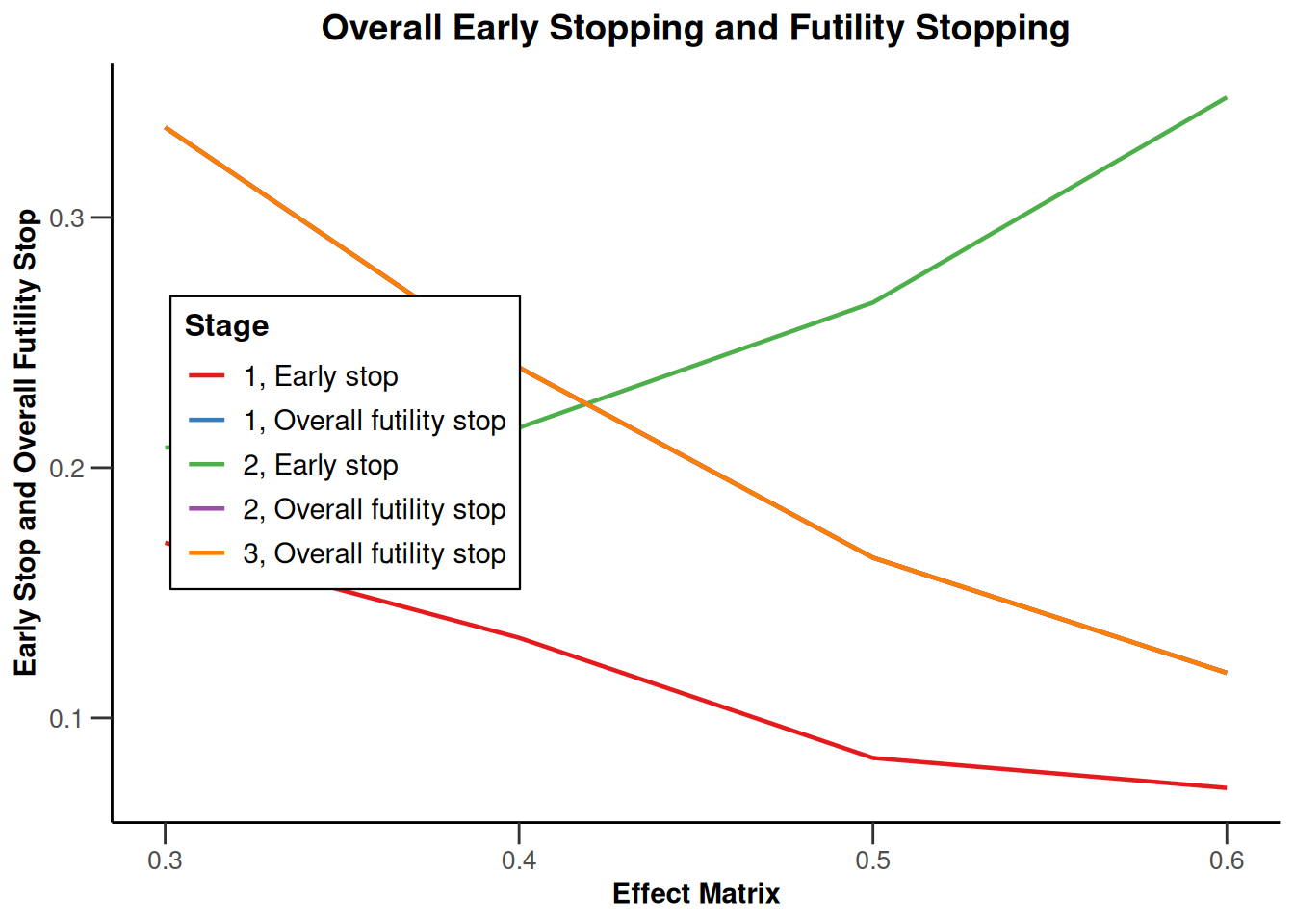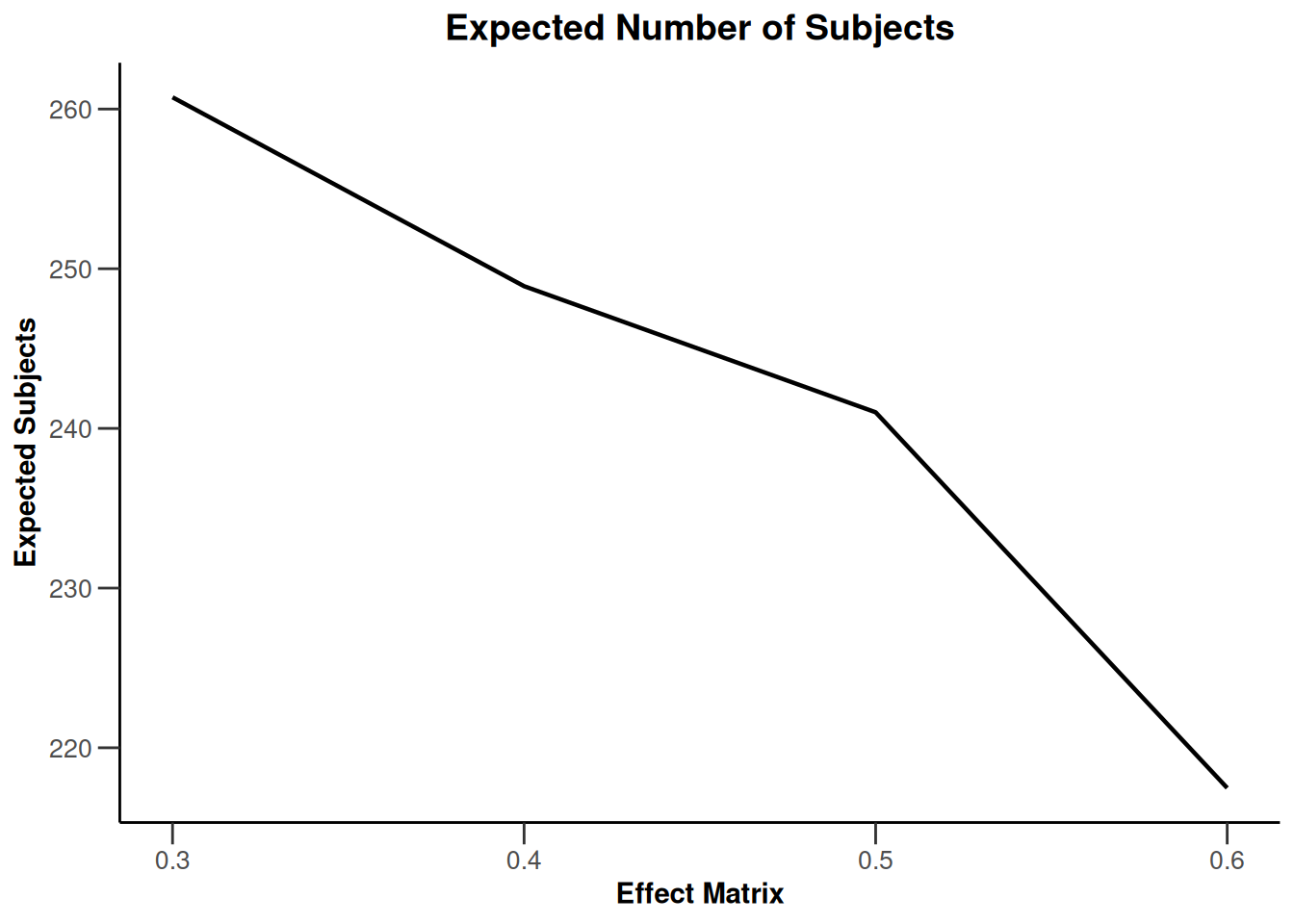## Simulation results multi-arm - rates

``````design <- getDesignInverseNormal(
informationRates = c(0.2, 0.6, 1),
futilityBounds = c(-0.5, 0.5)
)
x <- getSimulationMultiArmRates(
design = design, typeOfShape = "linear",
activeArms = 4, plannedSubjects = c(10, 30, 50),
piControl = 0.3, piMaxVector = seq(0.3, 0.6, 0.1),
adaptations = rep(TRUE, 2), conditionalPower = 0.8,
minNumberOfSubjectsPerStage = c(10, 4, 4),
maxNumberOfSubjectsPerStage = c(10, 100, 100),
maxNumberOfIterations = 500, seed = 1234567890
)
plot(x, type = "all", grid = 0)``````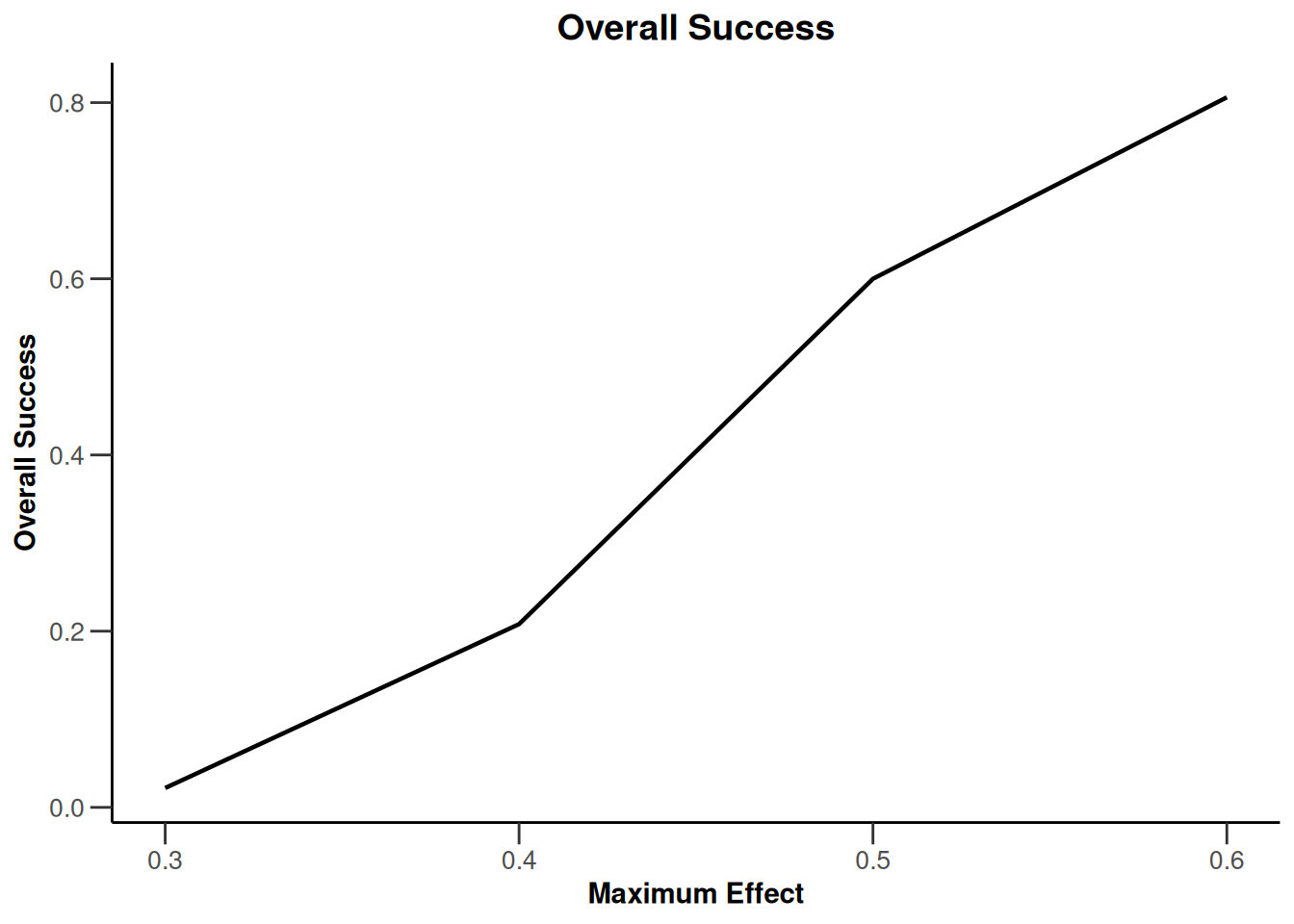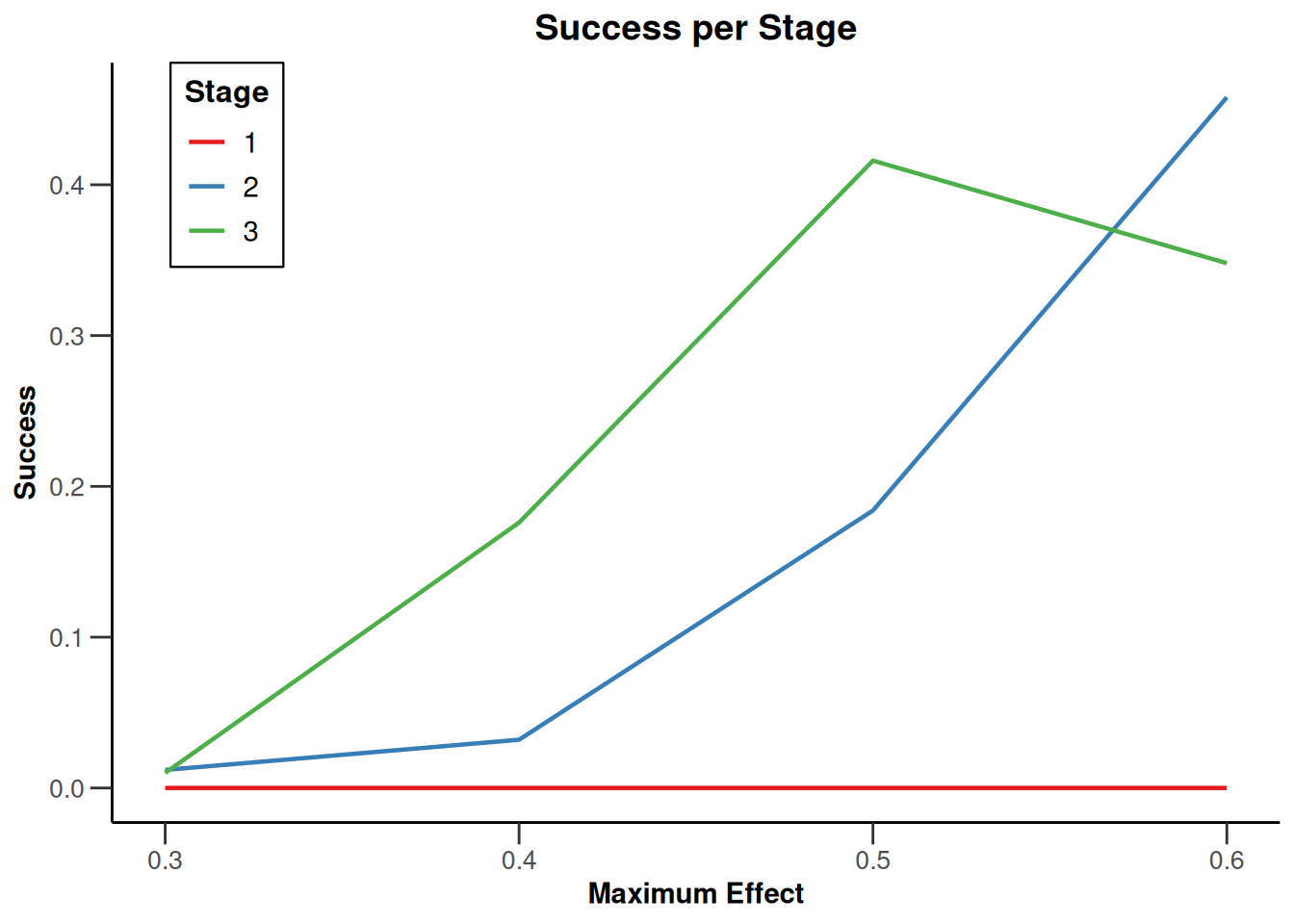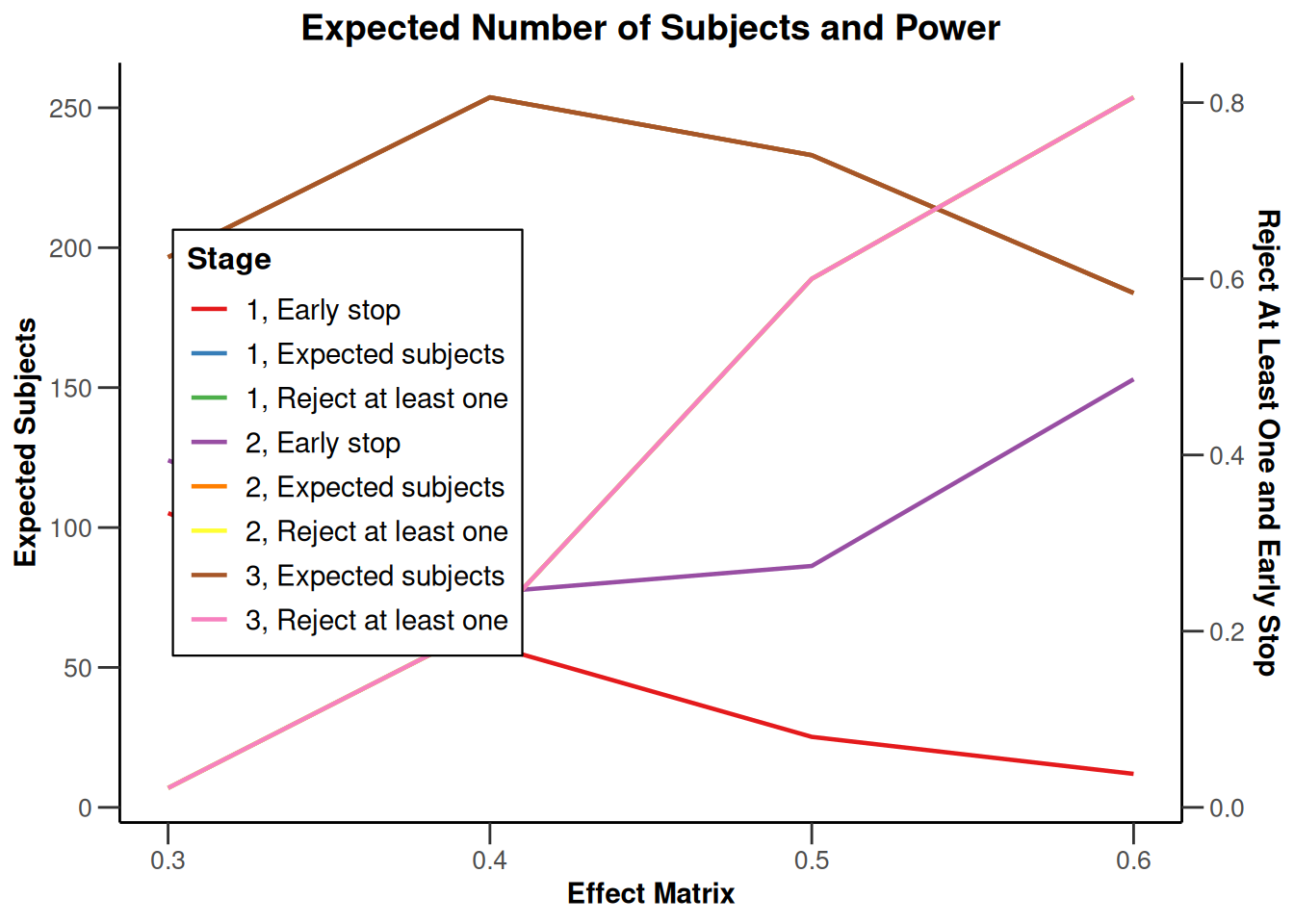## Simulation results multi-arm - survival

``````design <- getDesignInverseNormal(
informationRates = c(0.2, 0.6, 1),
futilityBounds = c(-0.5, 0.5)
)
x <- getSimulationMultiArmSurvival(
design = design, activeArms = 4,
typeOfSelection = "rBest", rValue = 2, plannedEvents = c(10, 30, 50),
omegaMaxVector = seq(1, 1.6, 0.2), adaptations = rep(TRUE, 2),
conditionalPower = 0.8, minNumberOfEventsPerStage = c(10, 4, 4),
maxNumberOfEventsPerStage = c(10, 100, 100),
maxNumberOfIterations = 500, seed = 1234567890
)
plot(x, type = "all", grid = 0)``````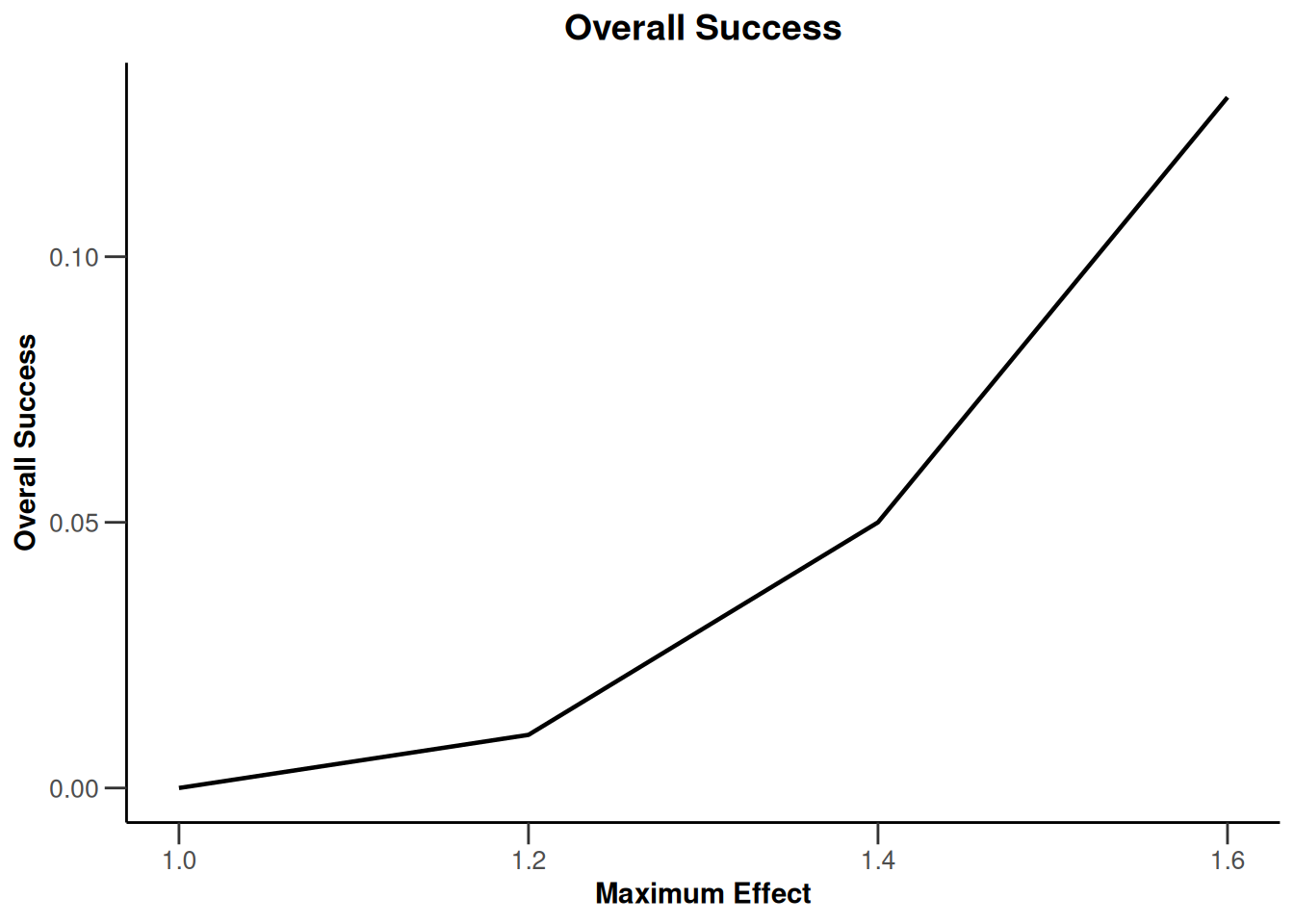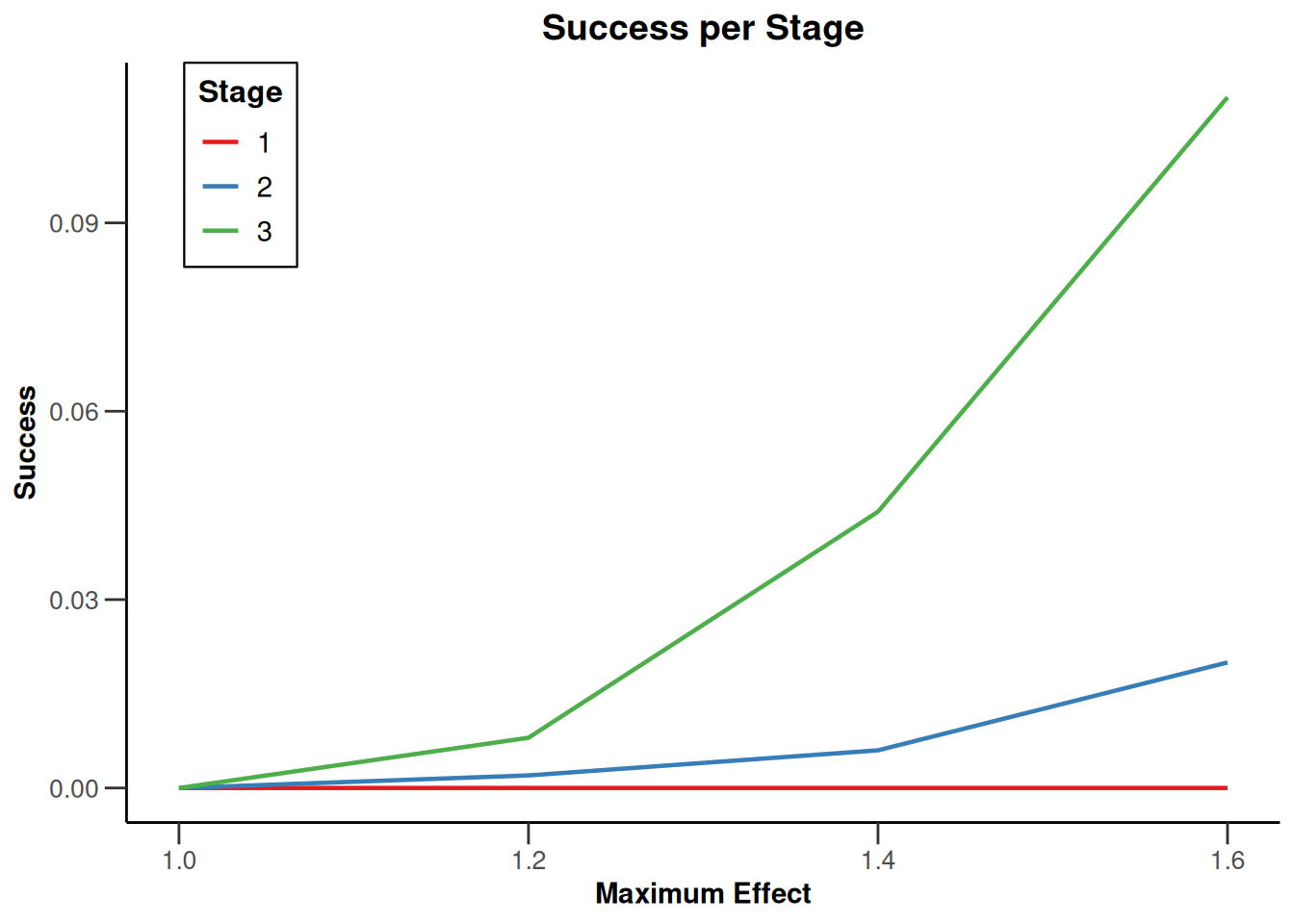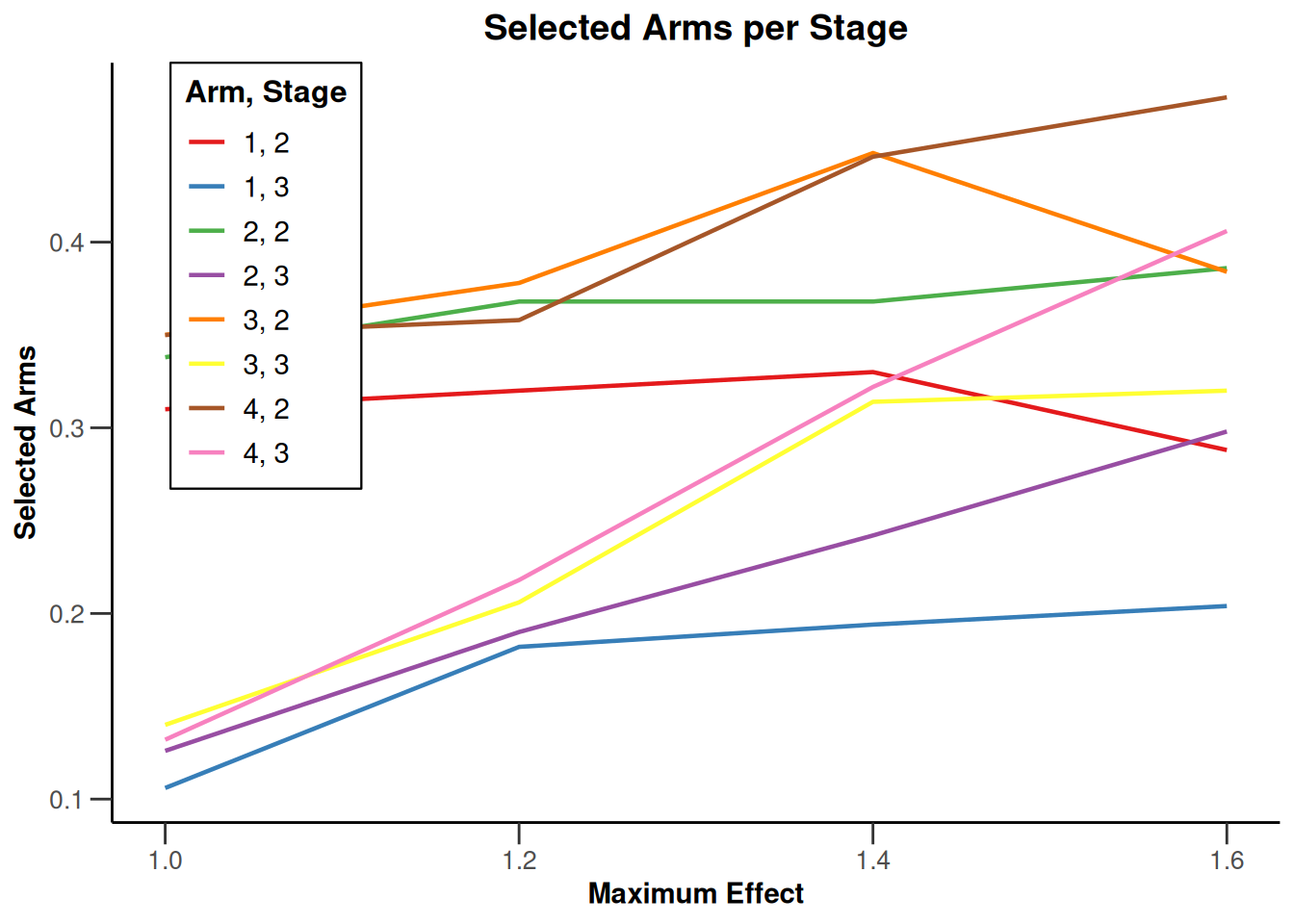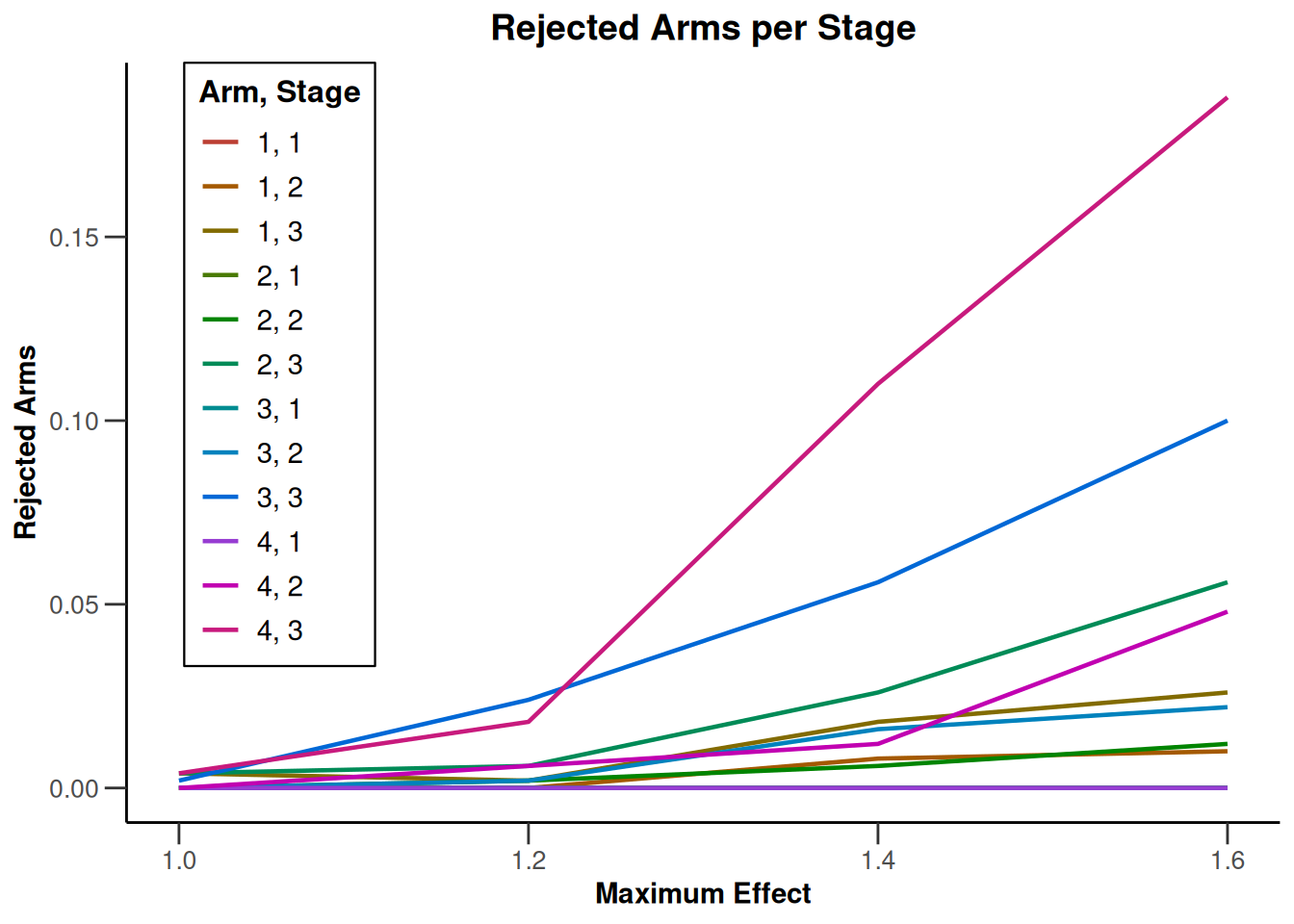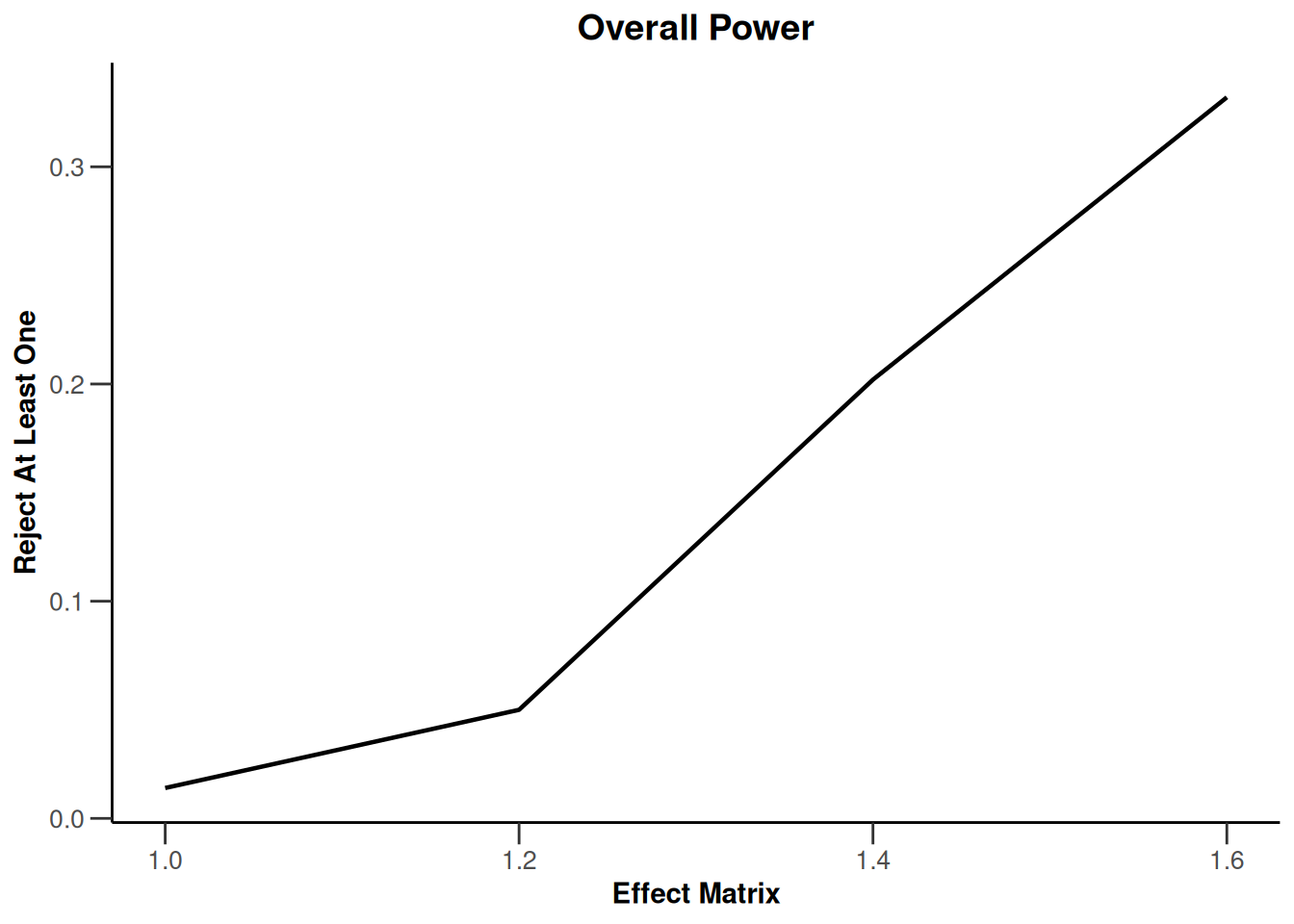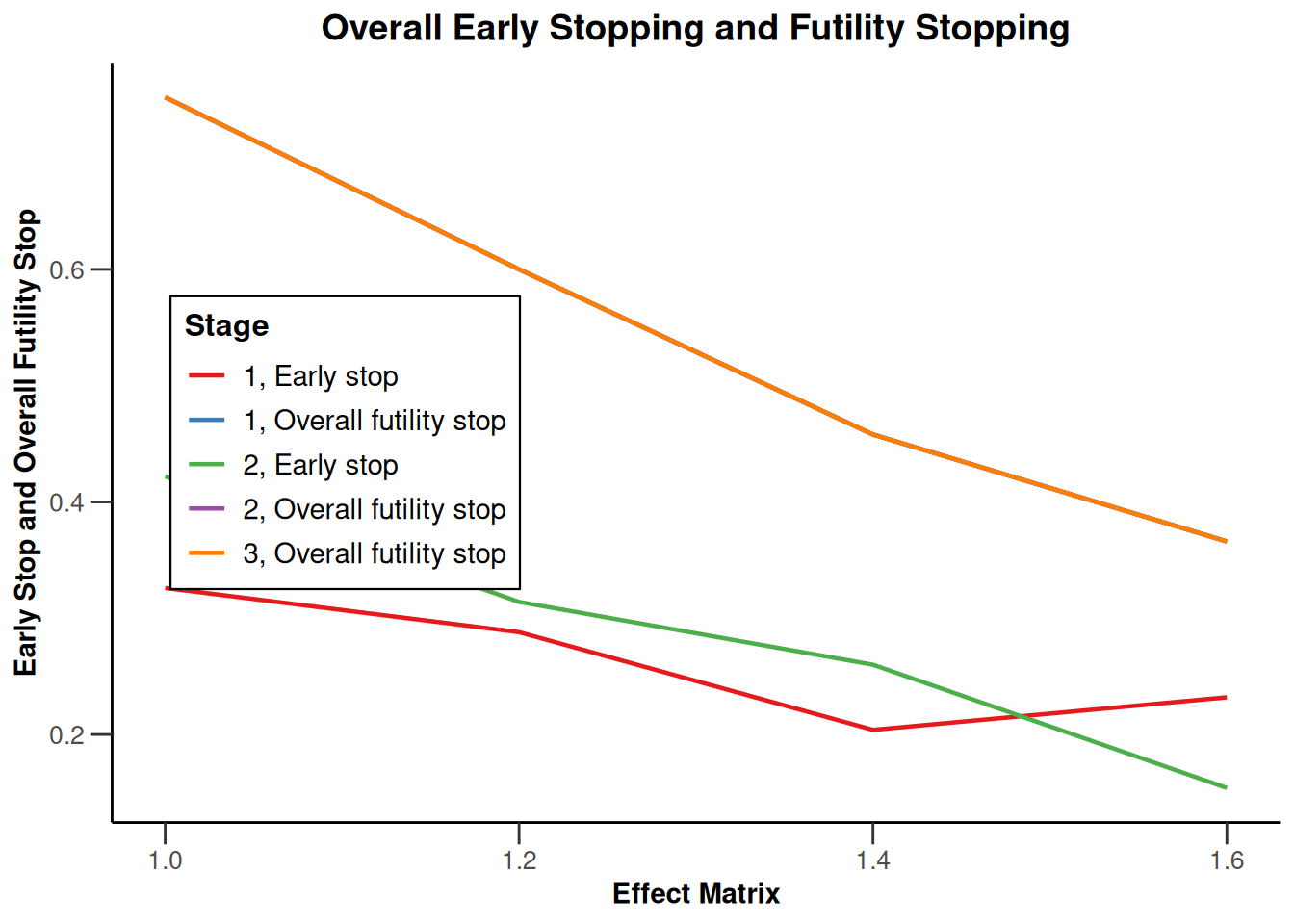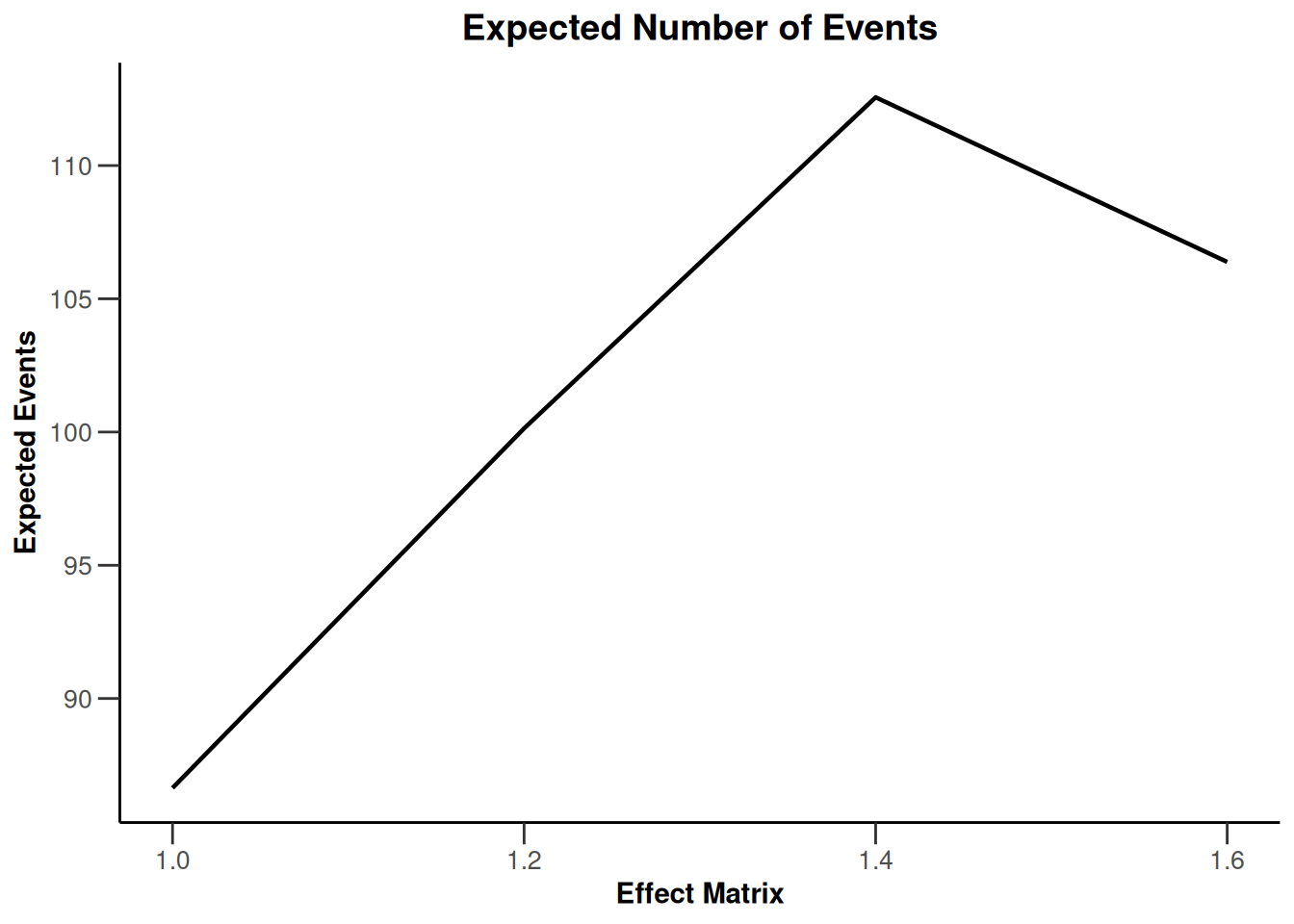# Simulation results enrichment

## Simulation results enrichment - means

``````design <- getDesignInverseNormal(
informationRates = c(0.2, 0.6, 1),
futilityBounds = c(-0.5, 0.5)
)
# Define subgroups and their prevalences
subGroups <- c("S1", "S12", "S2", "R") # fixed names!
prevalences <- c(0.2, 0.3, 0.4, 0.1)

effectR <- 1.5
effectS12 <- 5
m <- c()
for (effectS1 in seq(0, 5, 5)) {
for (effectS2 in seq(0, 5, 5)) {
m <- c(m, effectS1, effectS12, effectS2, effectR)
}
}
effects <- matrix(m, byrow = TRUE, ncol = 4)
stDev <- 10
# Define effect list
el <- list(
subGroups = subGroups, prevalences = prevalences,
stDevs = stDev, effects = effects
)

x <- getSimulationEnrichmentMeans(
design = design,
plannedSubjects = c(10, 30, 50),
effectList = el,
conditionalPower = 0.8,
minNumberOfSubjectsPerStage = c(10, 4, 4),
maxNumberOfSubjectsPerStage = c(10, 100, 100),
maxNumberOfIterations = 500,
seed = 1234567890
)``````
``````Warning: Simulation of enrichment designs is experimental and hence not fully
validated (see www.rpact.com/experimental)``````
``plot(x, type = "all", grid = 0)``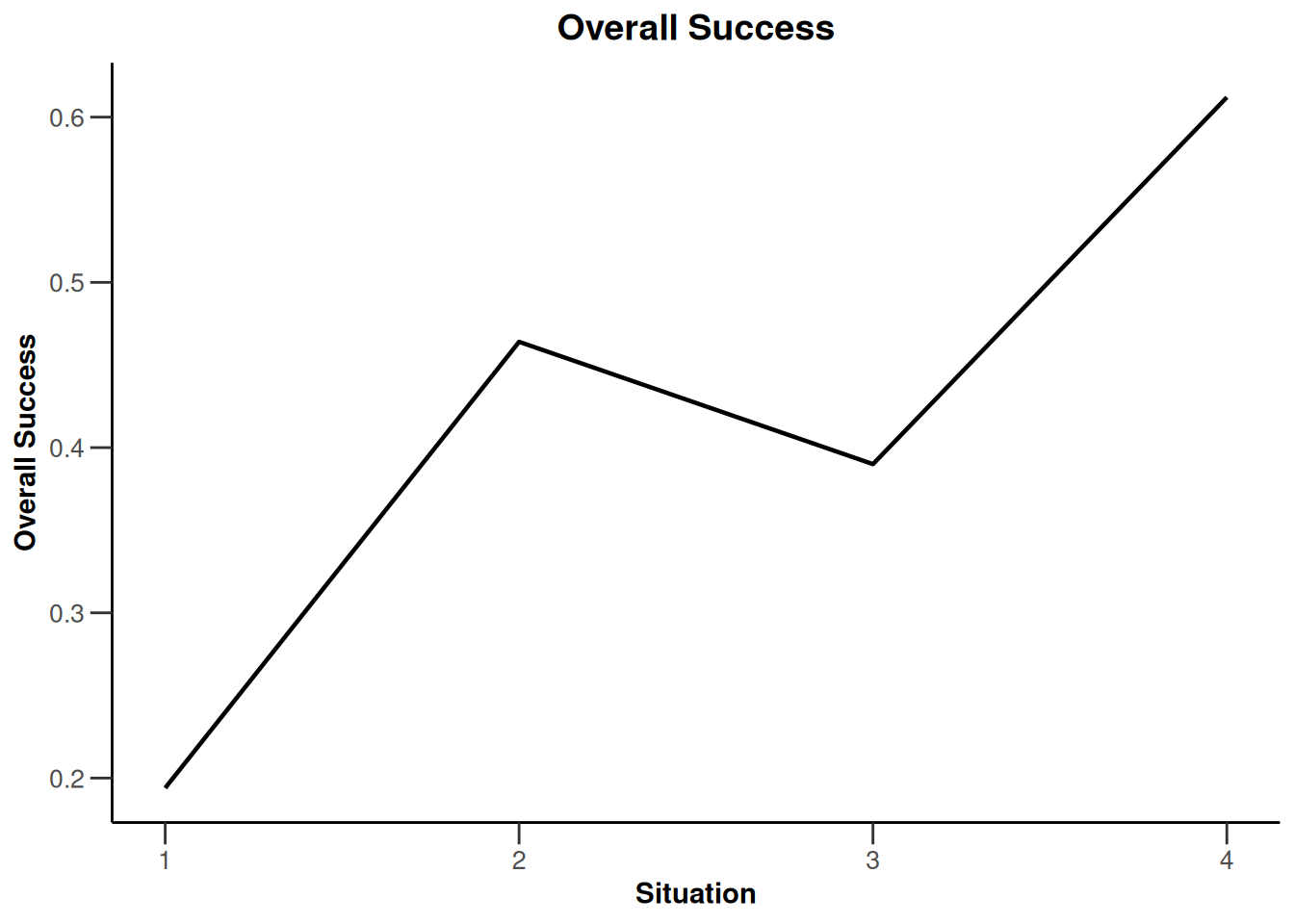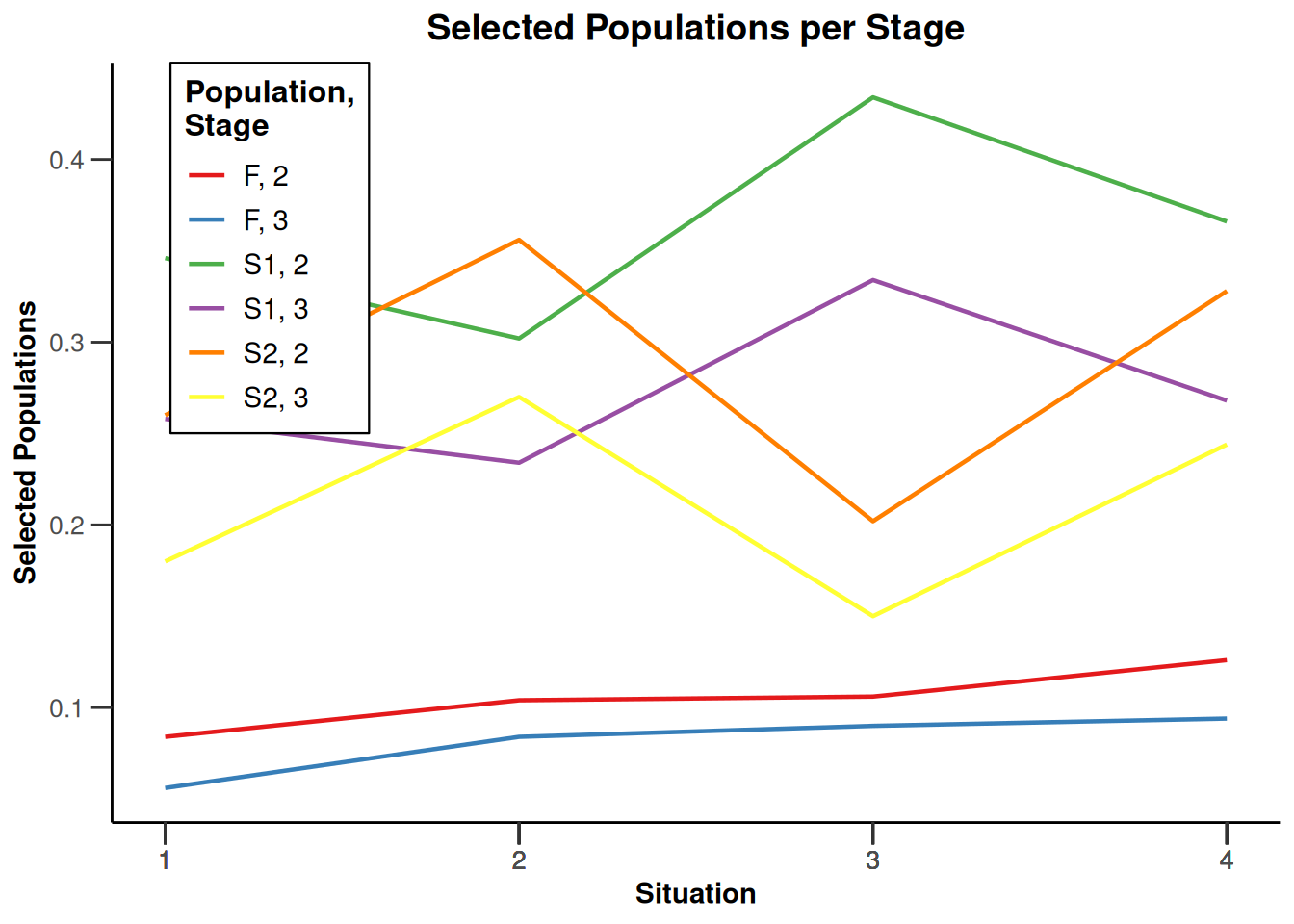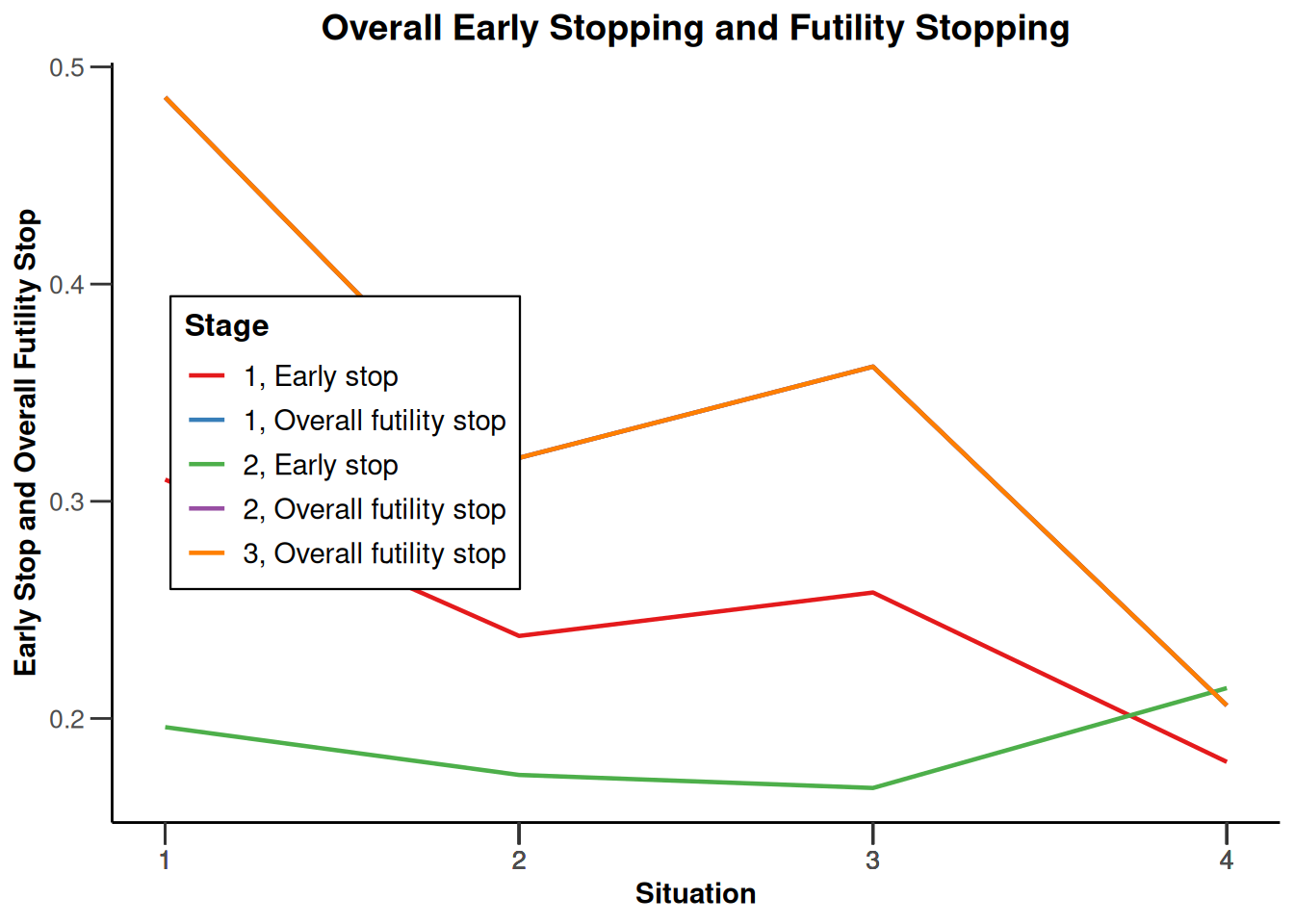## Simulation results enrichment - rates

``````design <- getDesignInverseNormal(
informationRates = c(0.2, 0.6, 1),
futilityBounds = c(-0.5, 0.5)
)
# Define effect list
subGroups <- c("S", "R")
prevalences <- c(0.4, 0.6)
piControl <- c(0.1, 0.4)
range1 <- piControl + seq(0.0, 0.2, 0.1)
range2 <- piControl + seq(0.0, 0.2, 0.1)
piTreatments <- c()
for (x1 in range1) {
for (x2 in range2) {
piTreatments <- c(piTreatments, x1, x2)
}
}
el <- list(
subGroups = subGroups,
prevalences = prevalences,
piControl = piControl,
piTreatments = matrix(piTreatments,
byrow = TRUE, ncol = 2
)
)

x <- getSimulationEnrichmentRates(
design = design,
plannedSubjects = c(10, 30, 50),
effectList = el,
maxNumberOfIterations = 500,
seed = 1234567890
)``````
``````Warning: Simulation of enrichment designs is experimental and hence not fully
validated (see www.rpact.com/experimental)``````
``plot(x, type = "all", grid = 0)``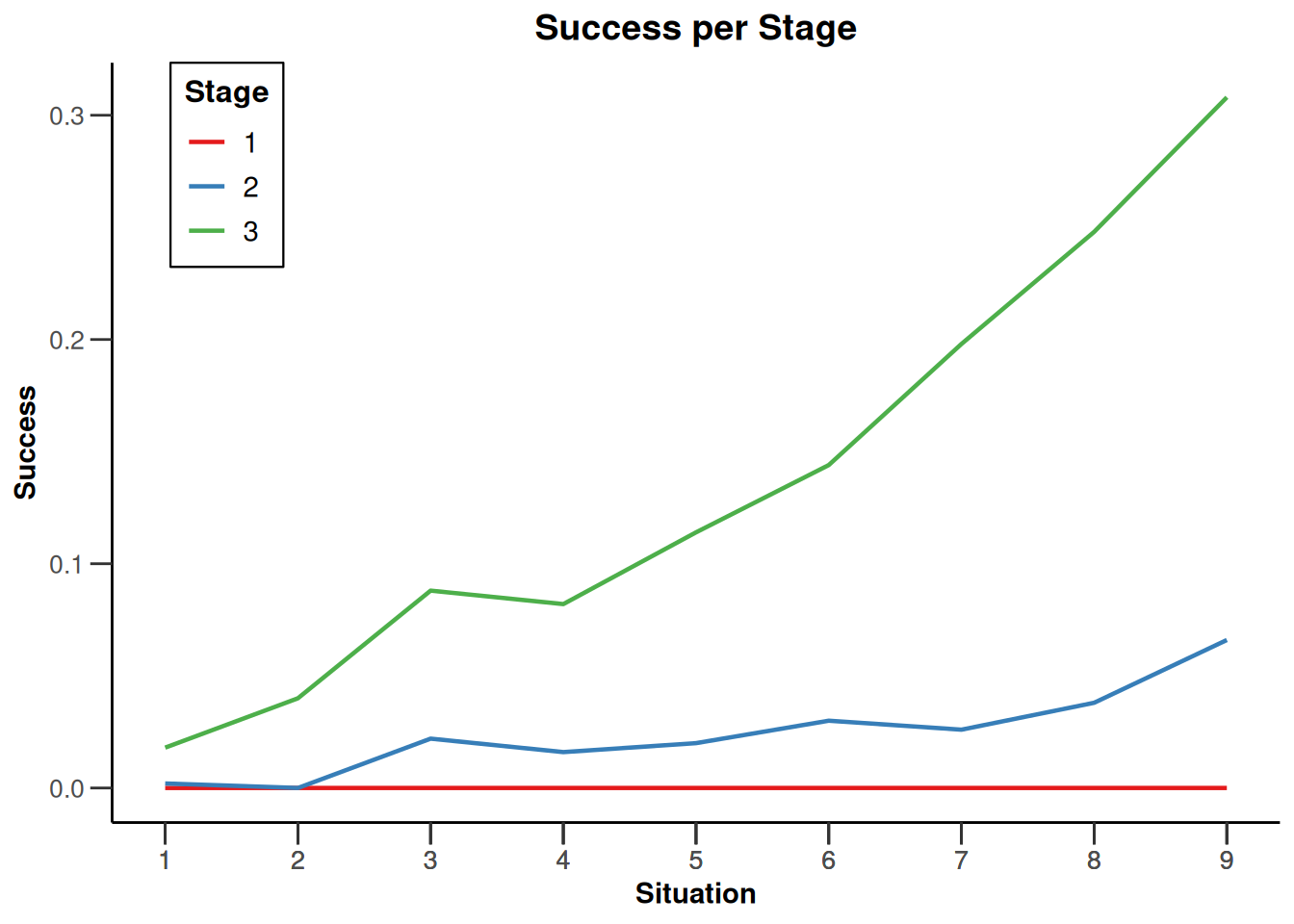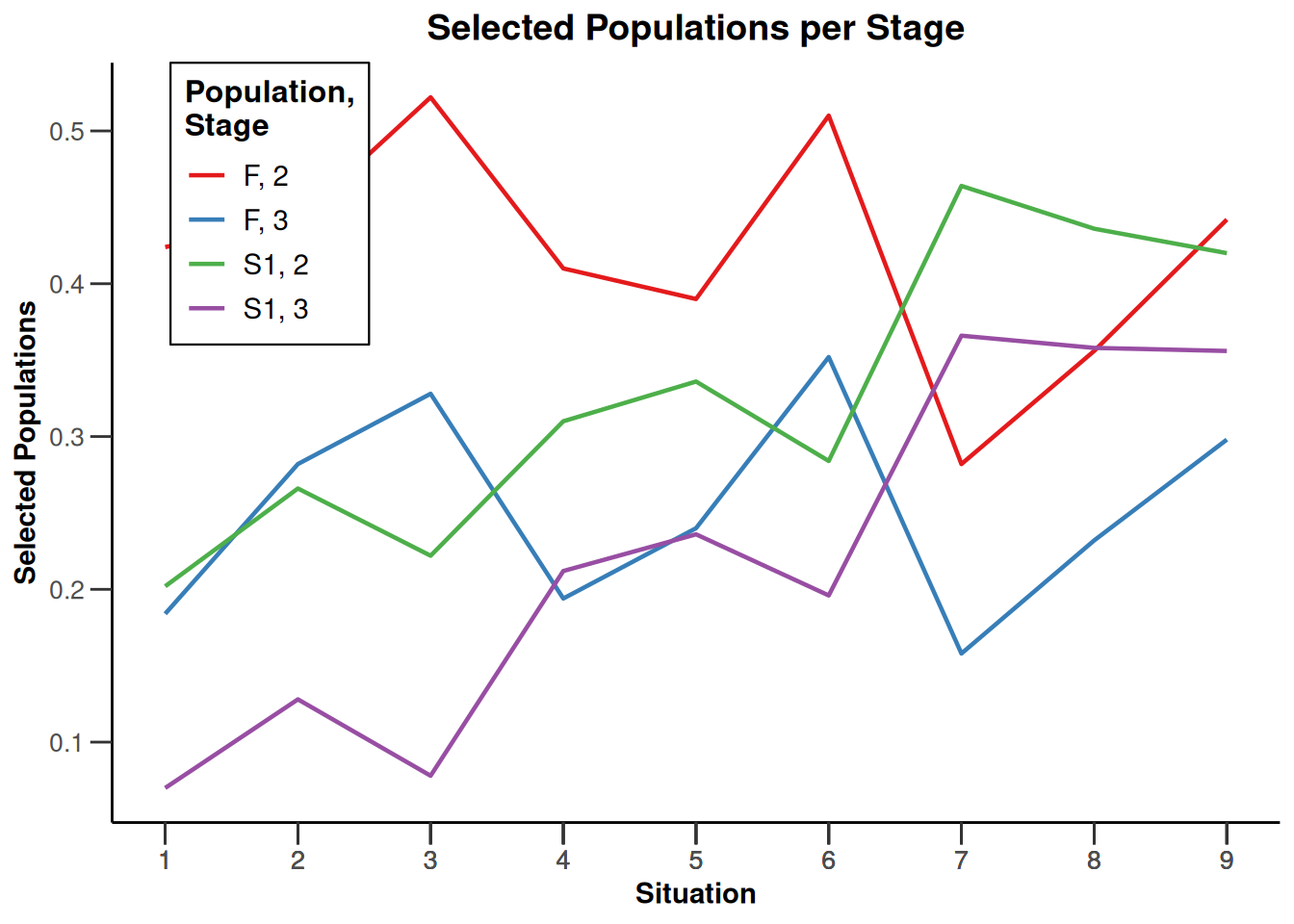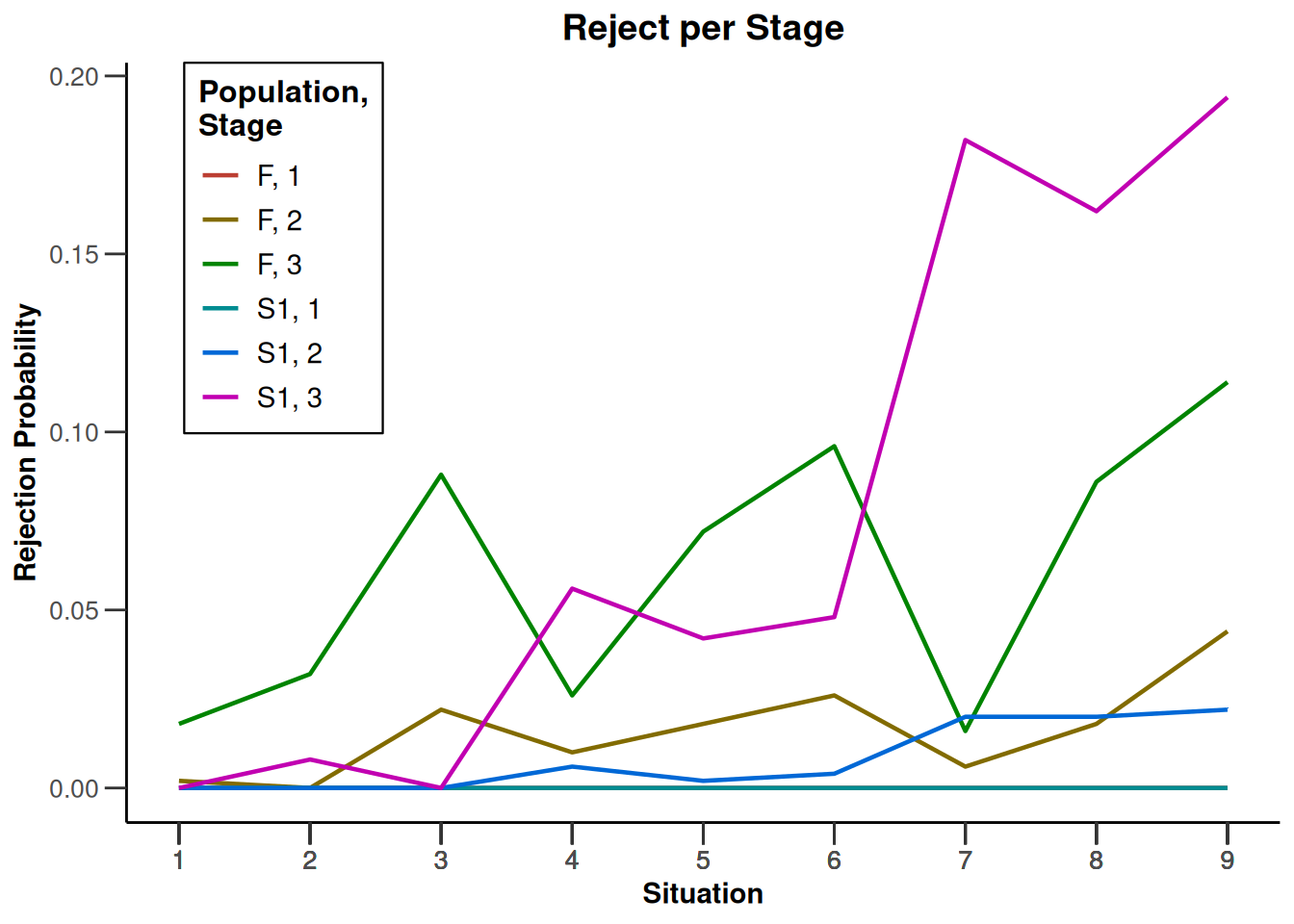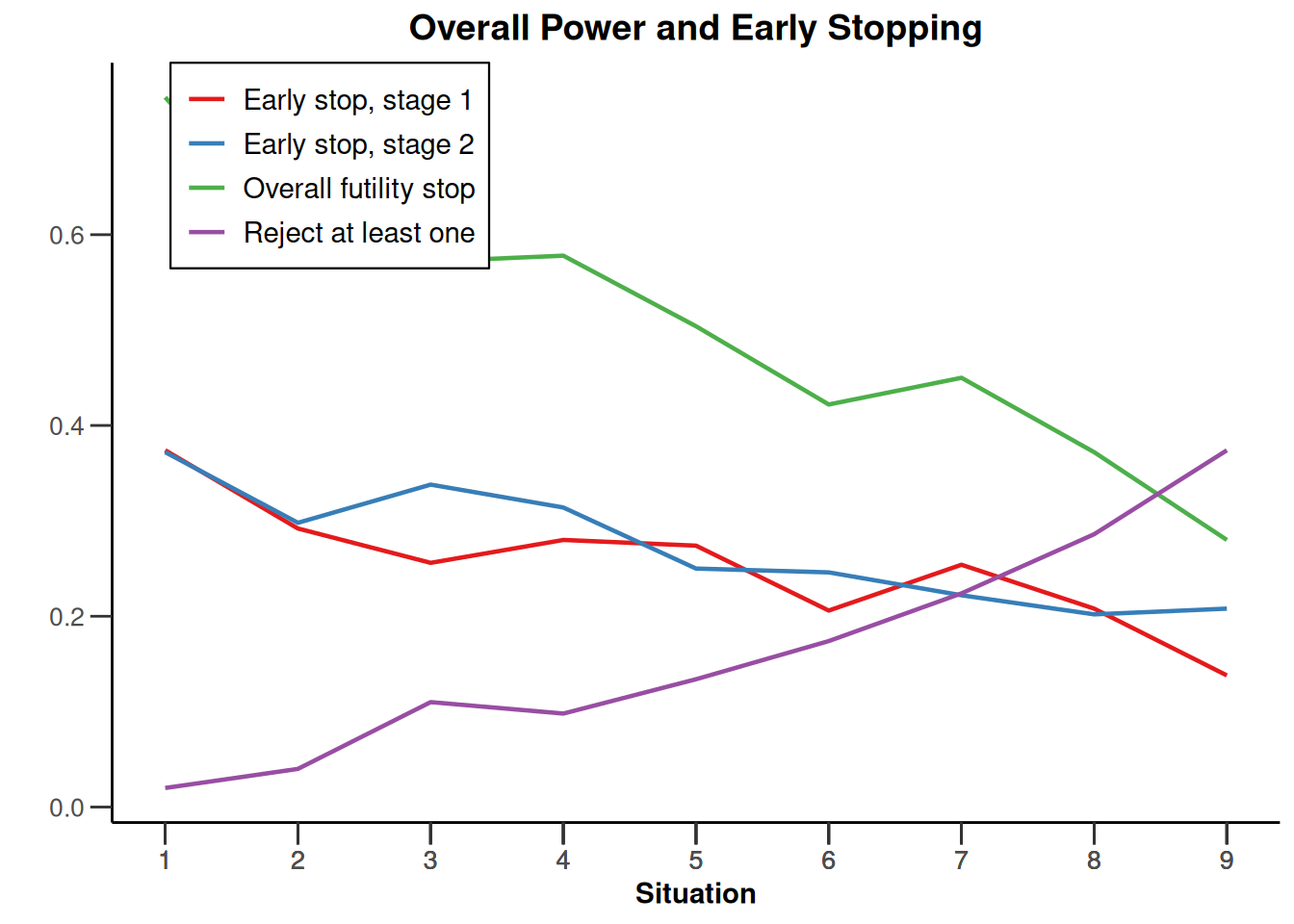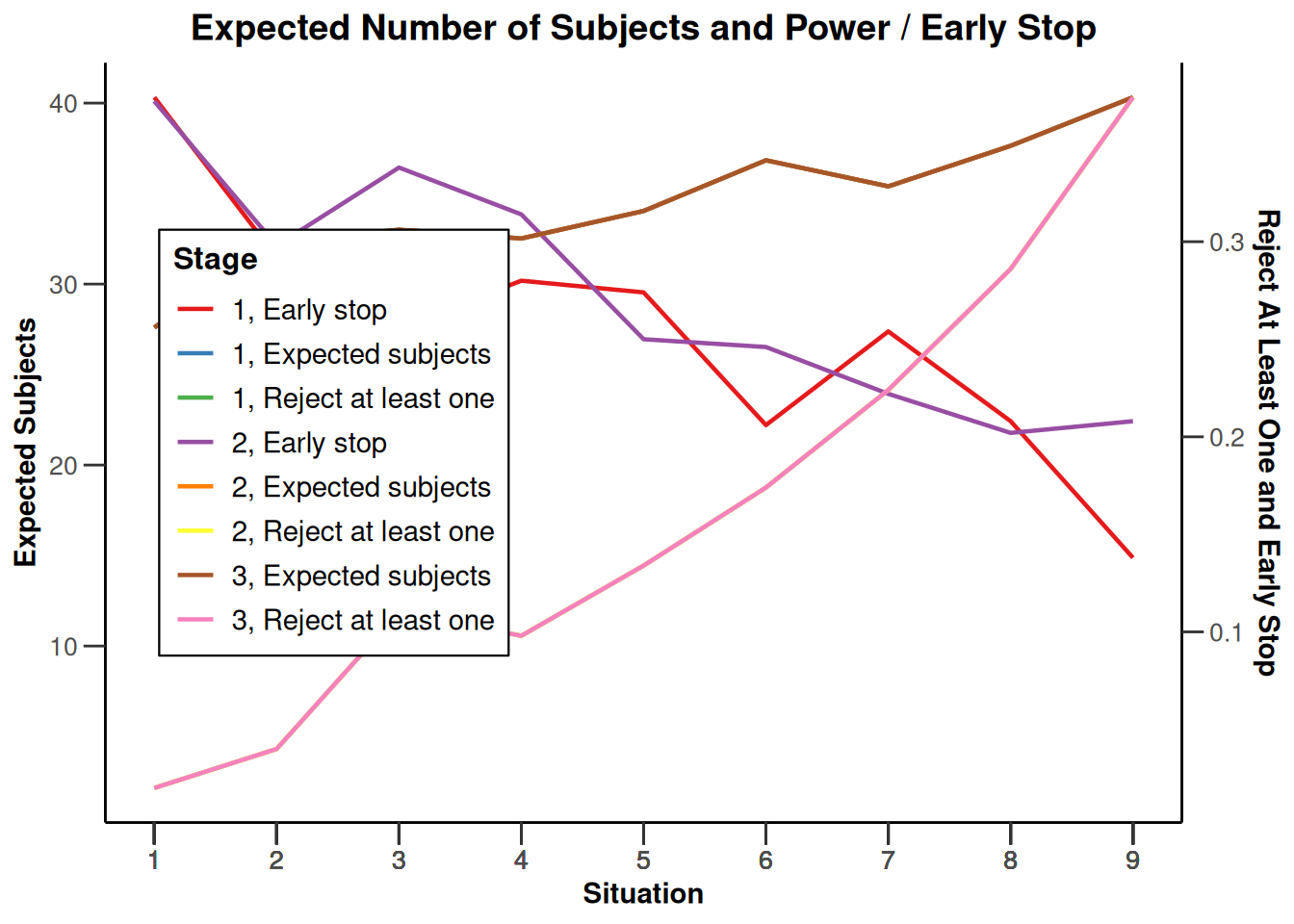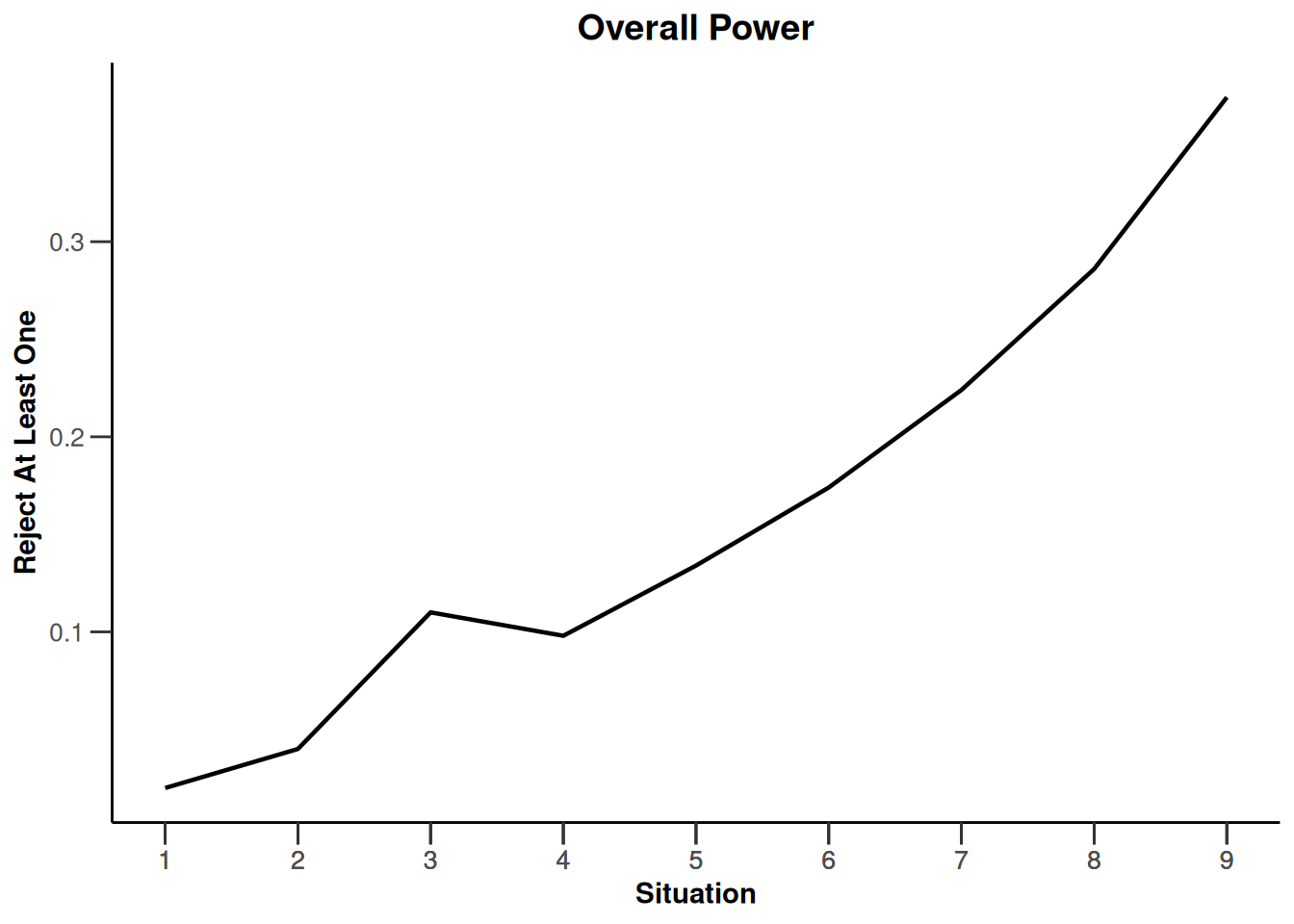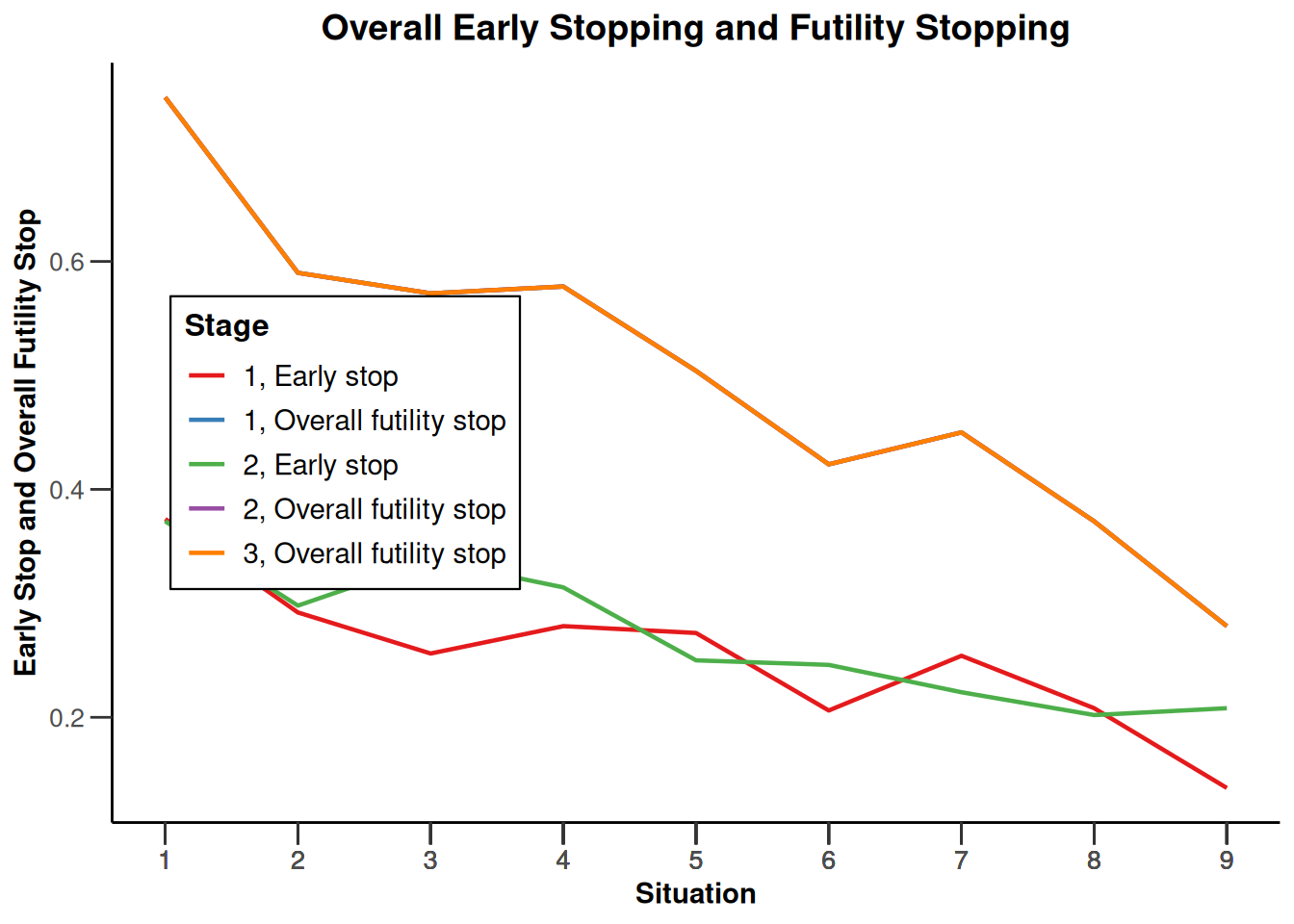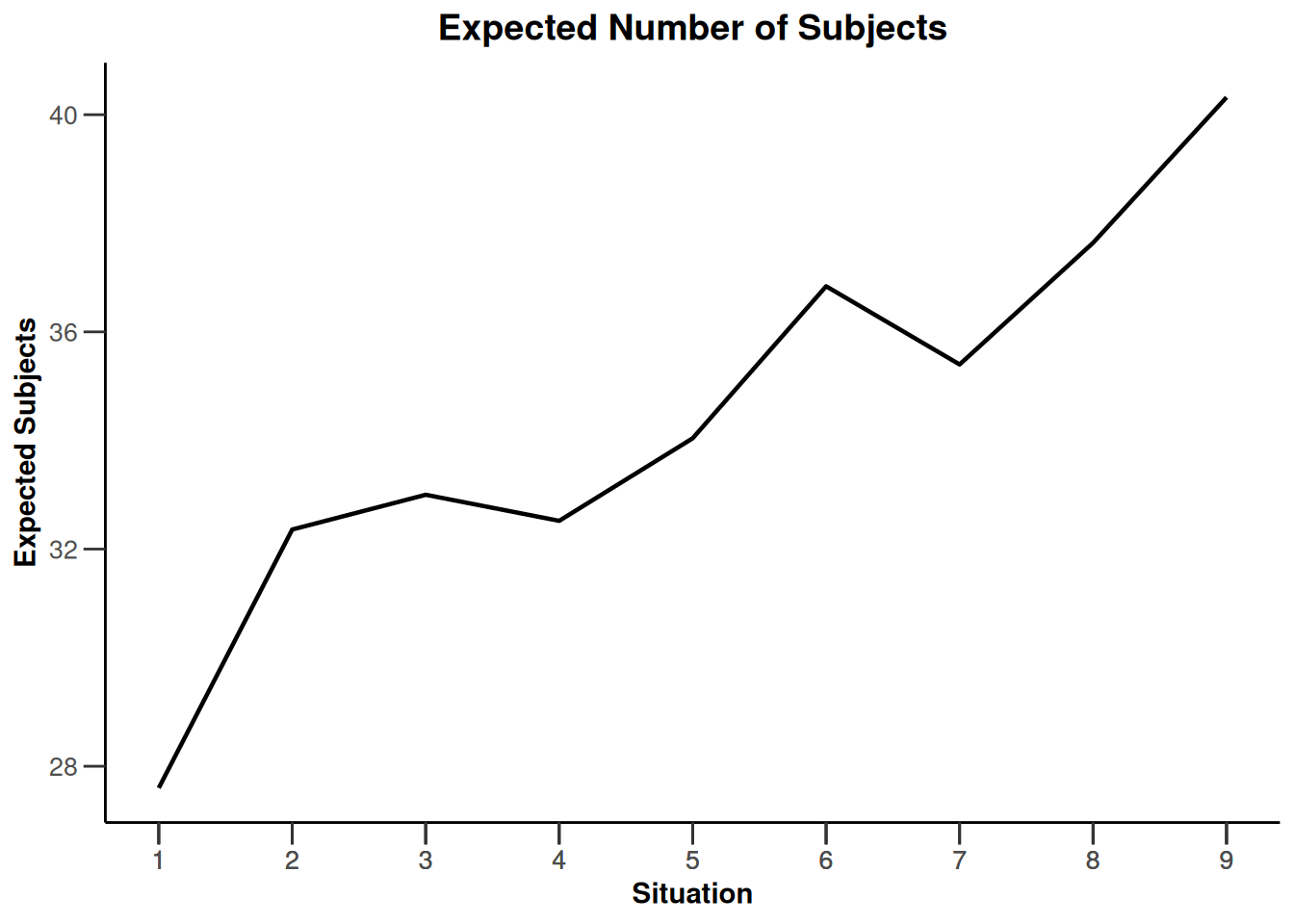## Simulation results enrichment - survival

``````# Define subgroups and their prevalences
subGroups <- c("S1", "S2", "S12", "R") # fixed names!
prevalences <- c(0.2, 0.3, 0.4, 0.1)

piControls <- c(0.2, 0.4, 0.15, 0.3)
effect <- c(-0.05, -0.02, -0.10, -0.10)
piTreatments <- piControls + effect

hr <- log(1 - piTreatments) / log(1 - piControls)

# Define effect list
el <- list(
subGroups = subGroups, prevalences = prevalences,
piControls = piControls, hazardRatios = matrix(rep(hr, 3), nrow = 3)
)

# Perform simulation
x <- getSimulationEnrichmentSurvival(
design = getDesignInverseNormal(typeOfDesign = "noEarlyEfficacy"),
effectList = el,
typeOfSelection = "rbest",
rValue = 2,
intersectionTest = "Simes",
plannedEvents = c(30, 80, 120),
maxNumberOfIterations = 500,
directionUpper = FALSE
)``````
``````Warning: Simulation of enrichment designs is experimental and hence not fully
validated (see www.rpact.com/experimental)``````
``plot(x, type = "all", grid = 0)``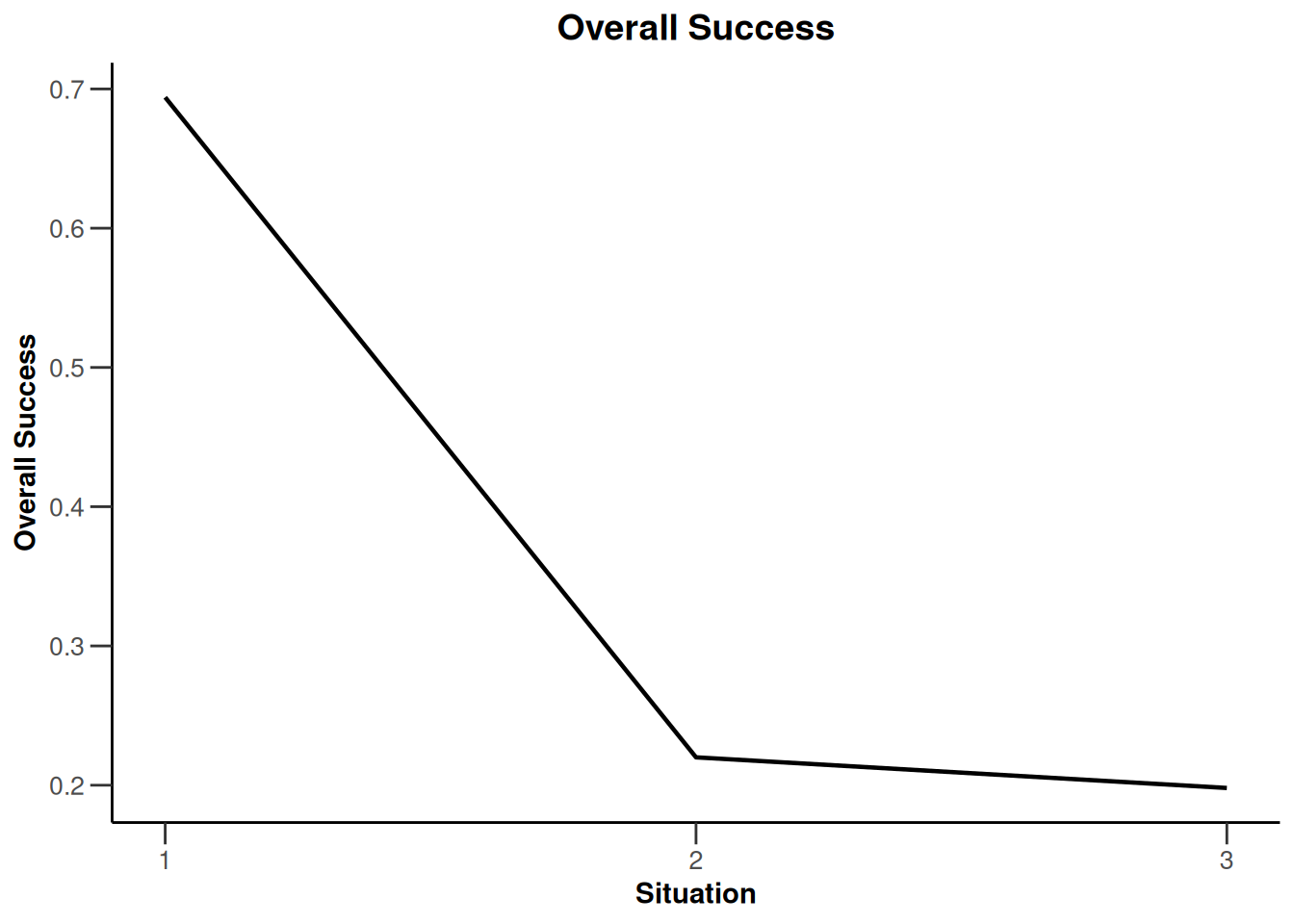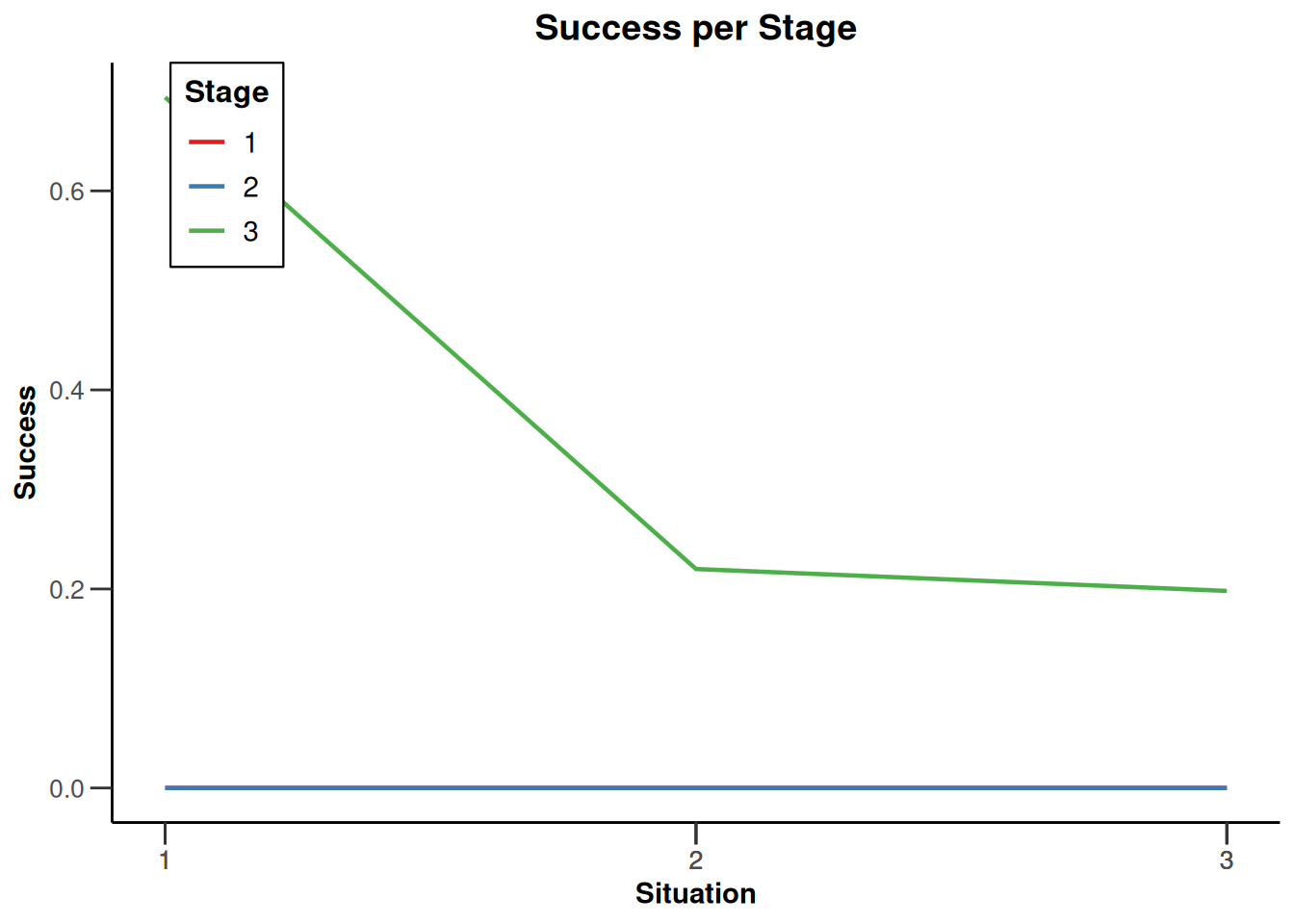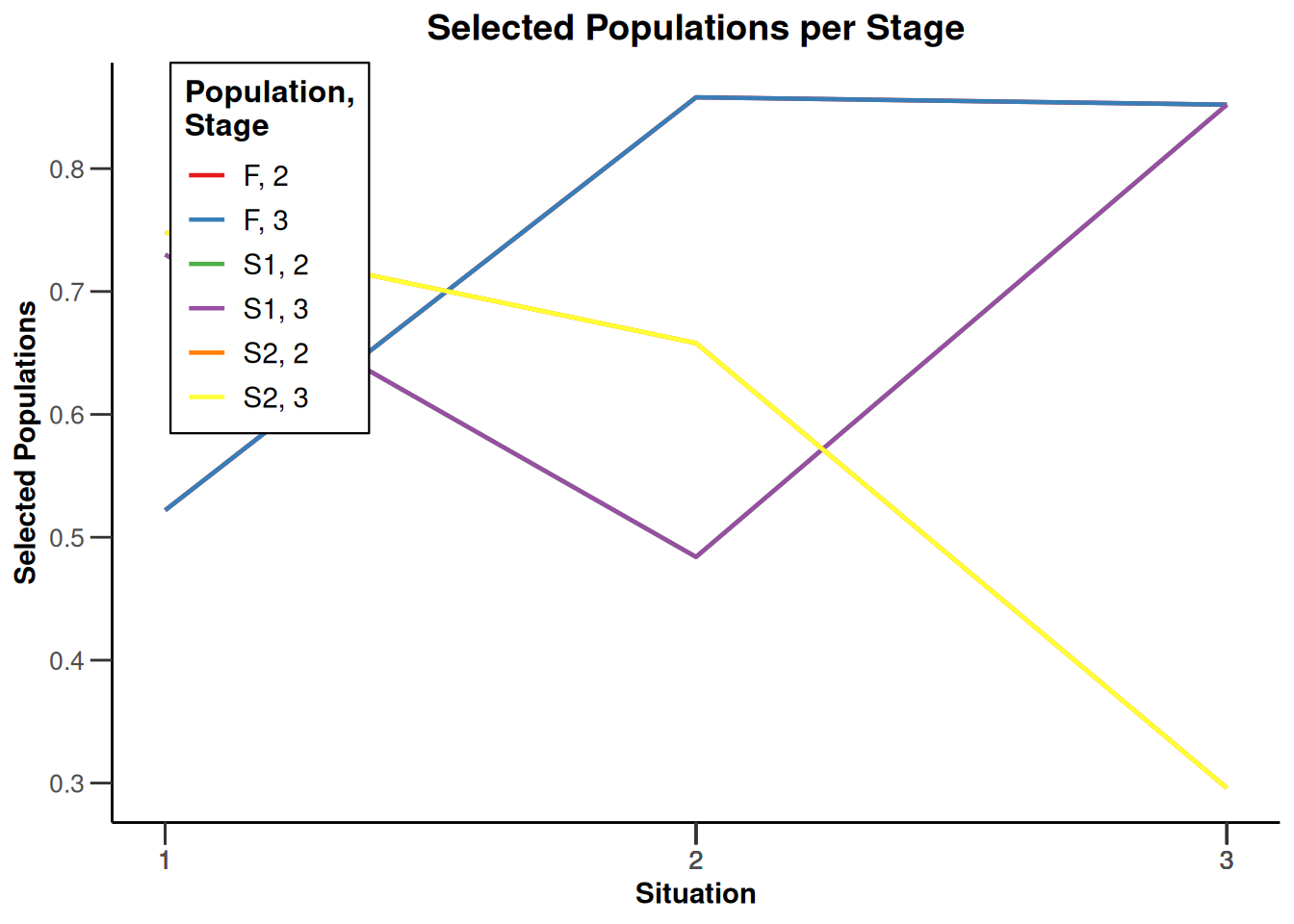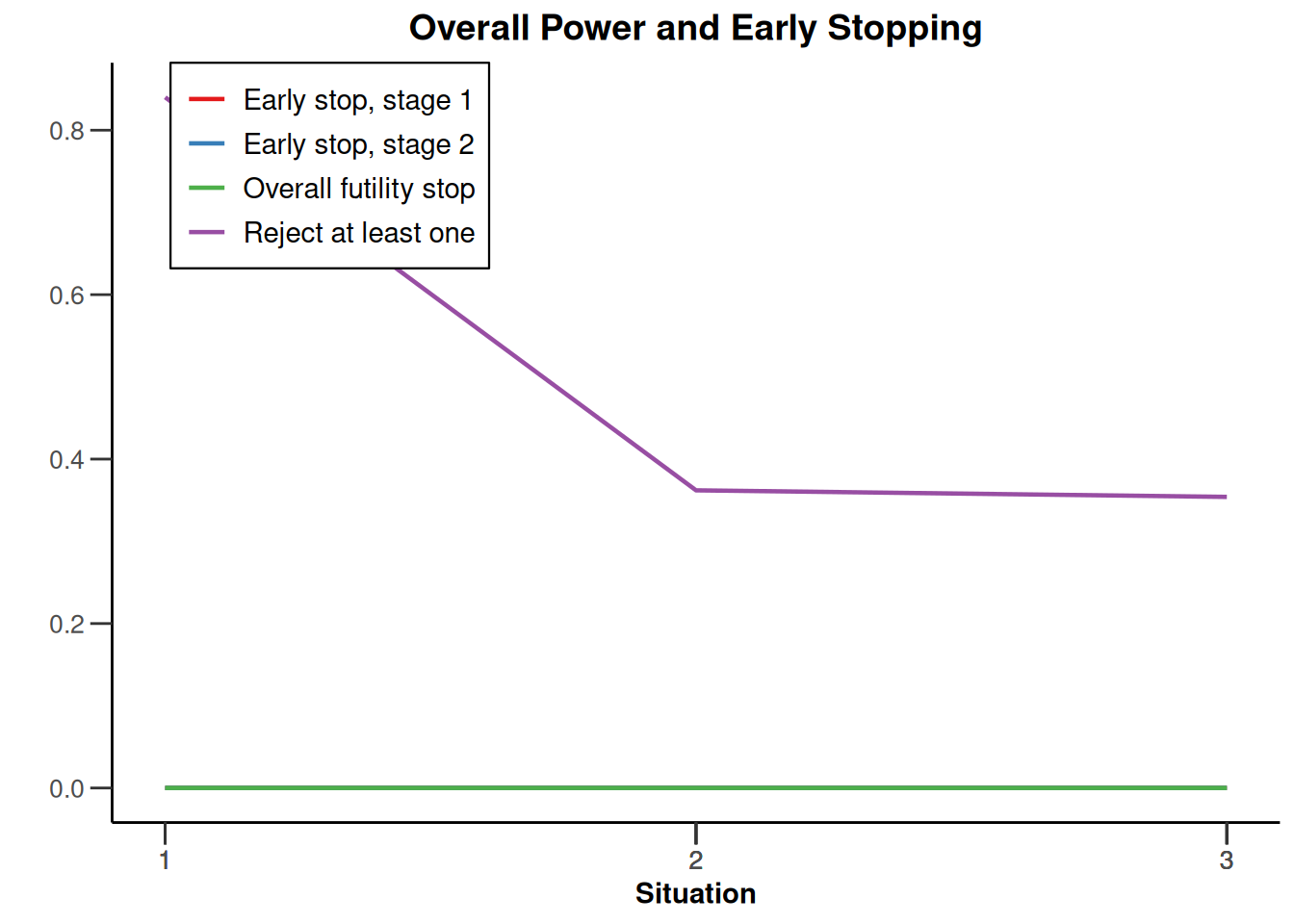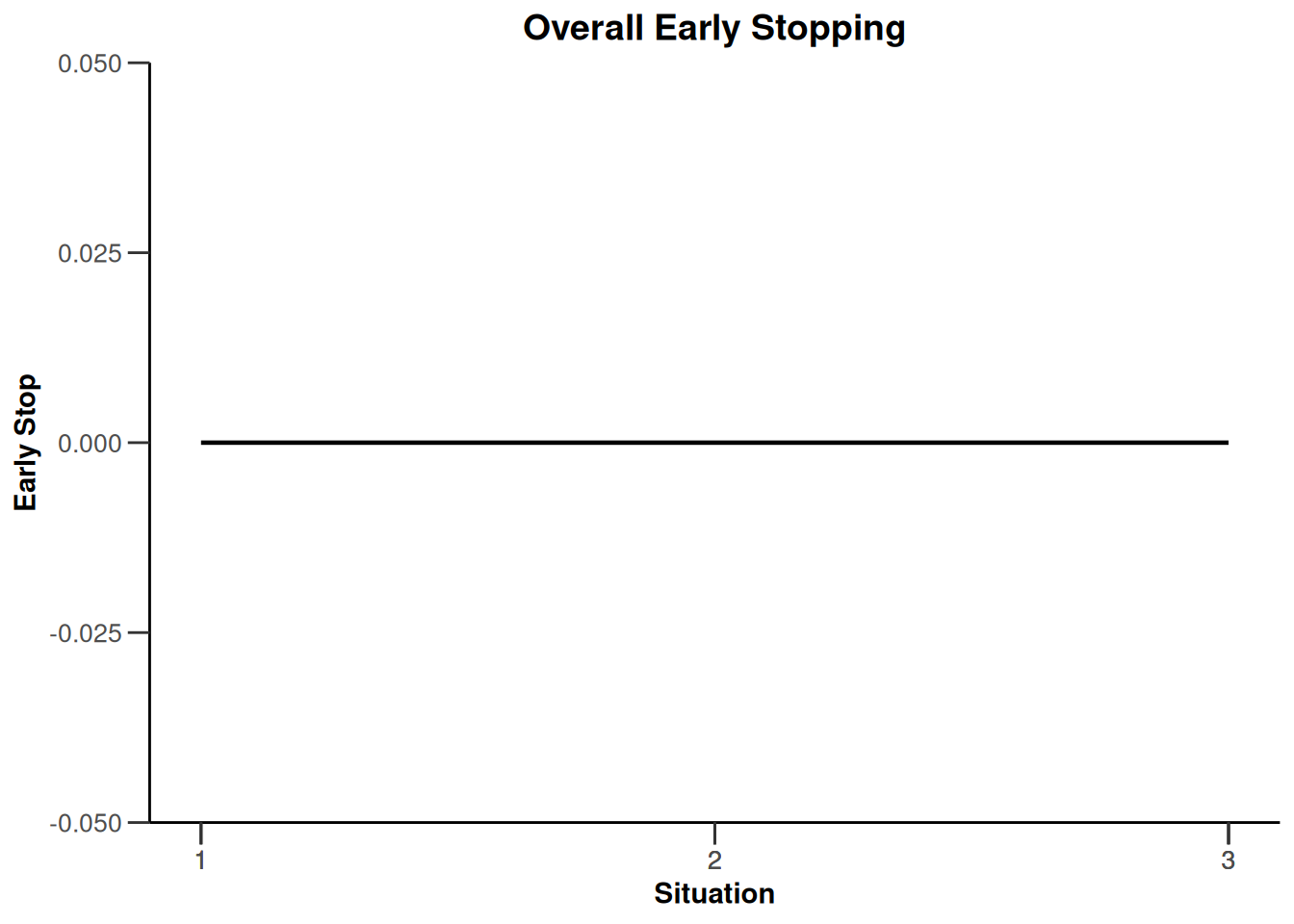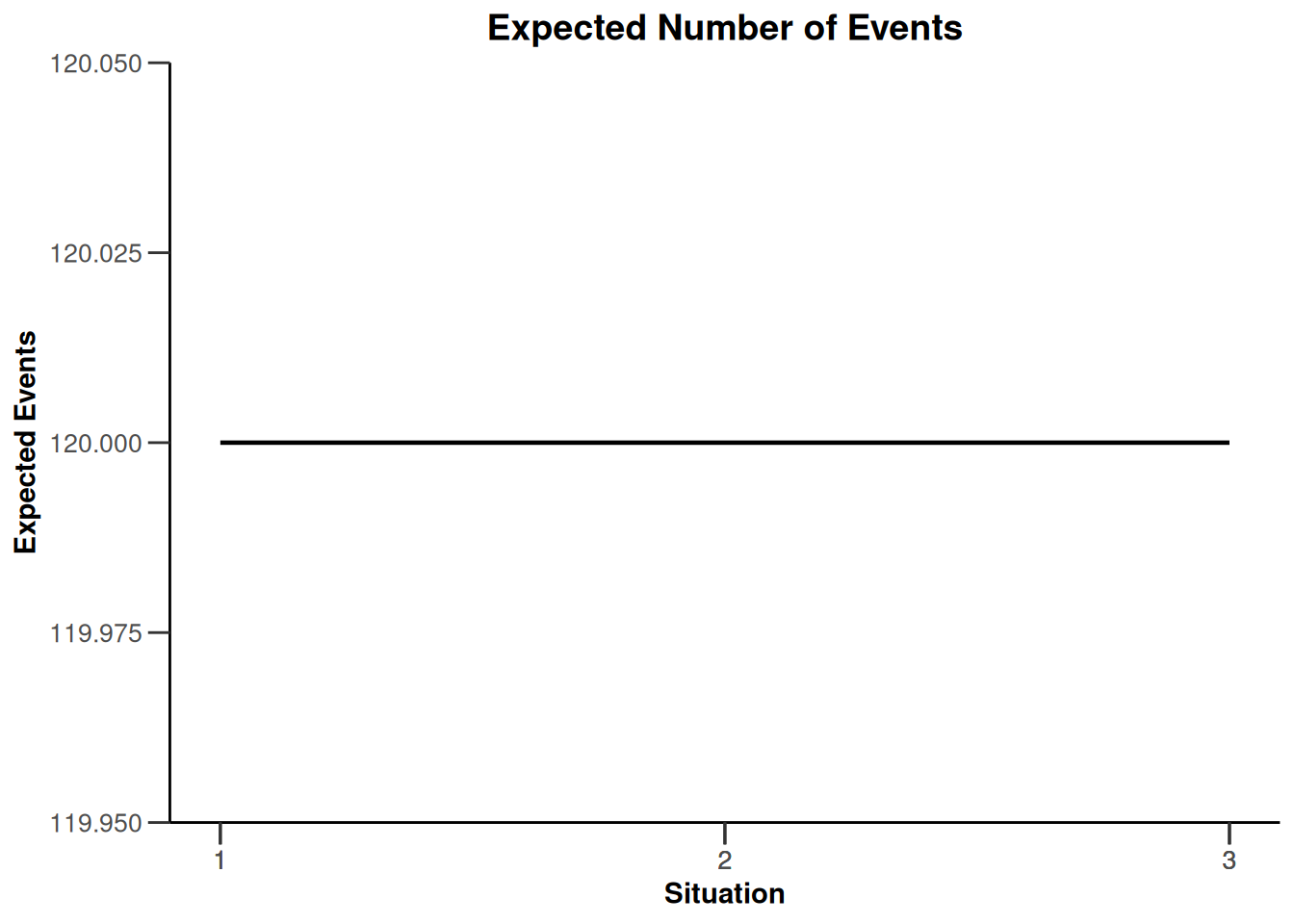System: rpact 3.3.4, R version 4.2.2 (2022-10-31 ucrt), platform: x86_64-w64-mingw32

To cite R in publications use:

R Core Team (2022). R: A language and environment for statistical computing. R Foundation for Statistical Computing, Vienna, Austria. URL https://www.R-project.org/.

To cite package ‘rpact’ in publications use:

Wassmer G, Pahlke F (2023). rpact: Confirmatory Adaptive Clinical Trial Design and Analysis. https://www.rpact.org, https://www.rpact.com, https://github.com/rpact-com/rpact.